# US7428693B2 - Error-detecting encoding and decoding apparatus and dividing apparatus - Google Patents

## Info

Publication number
US7428693B2
US7428693B2 US10/970,859 US97085904A US7428693B2 US 7428693 B2 US7428693 B2 US 7428693B2 US 97085904 A US97085904 A US 97085904A US 7428693 B2 US7428693 B2 US 7428693B2
Authority
US
United States
Prior art keywords
error
bit
data
input
sub
Prior art date
Legal status (The legal status is an assumption and is not a legal conclusion. Google has not performed a legal analysis and makes no representation as to the accuracy of the status listed.)
Expired - Fee Related, expires
Application number
US10/970,859
Other versions
US20050097432A1 (en
Inventor
Kazuhisa Obuchi
Tetsuya Yano
Takaharu Nakamura
Current Assignee (The listed assignees may be inaccurate. Google has not performed a legal analysis and makes no representation or warranty as to the accuracy of the list.)
Fujitsu Ltd
Original Assignee
Fujitsu Ltd
Priority date (The priority date is an assumption and is not a legal conclusion. Google has not performed a legal analysis and makes no representation as to the accuracy of the date listed.)
Filing date
Publication date
Priority to PCT/JP2002/003965 priority Critical patent/WO2003090362A1/en
Application filed by Fujitsu Ltd filed Critical Fujitsu Ltd
Assigned to FUJITSU LIMITED reassignment FUJITSU LIMITED ASSIGNMENT OF ASSIGNORS INTEREST (SEE DOCUMENT FOR DETAILS). Assignors: NAKAMURA, TAKAHARU, OBUCHI, KAZUHISA, YANO, TETSUYA
Publication of US20050097432A1 publication Critical patent/US20050097432A1/en
Application granted granted Critical
Publication of US7428693B2 publication Critical patent/US7428693B2/en
Expired - Fee Related legal-status Critical Current

• 238000006243 chemical reactions Methods 0.000 claims abstract description 63
• 230000011218 segmentation Effects 0.000 claims abstract description 28
• 239000011159 matrix materials Substances 0.000 claims description 70
• 230000001131 transforming Effects 0.000 claims description 23
• 238000010586 diagrams Methods 0.000 description 86
• 244000241601 filaree Species 0.000 description 46
• 230000000694 effects Effects 0.000 description 33
• 239000010410 layers Substances 0.000 description 22
• 230000000875 corresponding Effects 0.000 description 4
• 239000000203 mixtures Substances 0.000 description 3
• 230000005540 biological transmission Effects 0.000 description 2
• 125000004122 cyclic group Chemical group 0.000 description 2
• 238000004891 communication Methods 0.000 description 1
• 239000000284 extracts Substances 0.000 description 1
• 230000002045 lasting Effects 0.000 description 1
• 238000010295 mobile communication Methods 0.000 description 1

## Images

•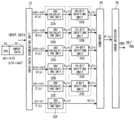•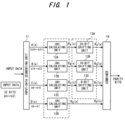••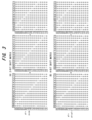••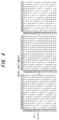•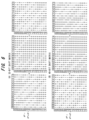•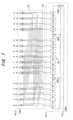••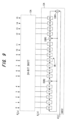••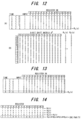••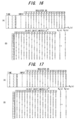•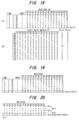•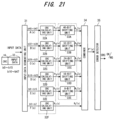•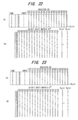•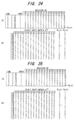••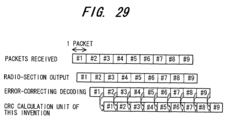•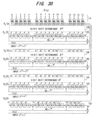•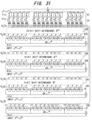•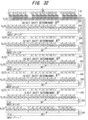•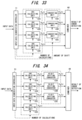•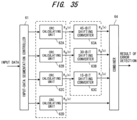••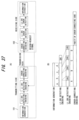•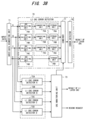•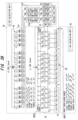•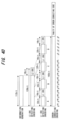•••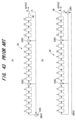•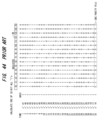•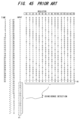•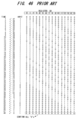•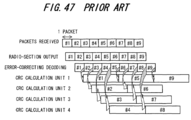## Classifications

• HELECTRICITY
• H03BASIC ELECTRONIC CIRCUITRY
• H03MCODING; DECODING; CODE CONVERSION IN GENERAL
• H03M13/00Coding, decoding or code conversion, for error detection or error correction; Coding theory basic assumptions; Coding bounds; Error probability evaluation methods; Channel models; Simulation or testing of codes
• H03M13/03Error detection or forward error correction by redundancy in data representation, i.e. code words containing more digits than the source words
• H03M13/05Error detection or forward error correction by redundancy in data representation, i.e. code words containing more digits than the source words using block codes, i.e. a predetermined number of check bits joined to a predetermined number of information bits
• H03M13/09Error detection only, e.g. using cyclic redundancy check [CRC] codes or single parity bit
• H03M13/091Parallel or block-wise CRC computation
• HELECTRICITY
• H03BASIC ELECTRONIC CIRCUITRY
• H03MCODING; DECODING; CODE CONVERSION IN GENERAL
• H03M13/00Coding, decoding or code conversion, for error detection or error correction; Coding theory basic assumptions; Coding bounds; Error probability evaluation methods; Channel models; Simulation or testing of codes
• H03M13/03Error detection or forward error correction by redundancy in data representation, i.e. code words containing more digits than the source words
• H03M13/05Error detection or forward error correction by redundancy in data representation, i.e. code words containing more digits than the source words using block codes, i.e. a predetermined number of check bits joined to a predetermined number of information bits
• H03M13/09Error detection only, e.g. using cyclic redundancy check [CRC] codes or single parity bit
• H03M13/093CRC update after modification of the information word
• HELECTRICITY
• H04ELECTRIC COMMUNICATION TECHNIQUE
• H04LTRANSMISSION OF DIGITAL INFORMATION, e.g. TELEGRAPHIC COMMUNICATION
• H04L1/00Arrangements for detecting or preventing errors in the information received
• H04L1/004Arrangements for detecting or preventing errors in the information received by using forward error control
• H04L1/0041Arrangements at the transmitter end
• H04L1/0043Realisations of complexity reduction techniques, e.g. use of look-up tables
• HELECTRICITY
• H04ELECTRIC COMMUNICATION TECHNIQUE
• H04LTRANSMISSION OF DIGITAL INFORMATION, e.g. TELEGRAPHIC COMMUNICATION
• H04L1/00Arrangements for detecting or preventing errors in the information received
• H04L1/004Arrangements for detecting or preventing errors in the information received by using forward error control
• H04L1/0056Systems characterized by the type of code used
• H04L1/0061Error detection codes
• HELECTRICITY
• H04ELECTRIC COMMUNICATION TECHNIQUE
• H04LTRANSMISSION OF DIGITAL INFORMATION, e.g. TELEGRAPHIC COMMUNICATION
• H04L1/00Arrangements for detecting or preventing errors in the information received
• H04L1/12Arrangements for detecting or preventing errors in the information received by using return channel
• H04L1/16Arrangements for detecting or preventing errors in the information received by using return channel in which the return channel carries supervisory signals, e.g. repetition request signals
• H04L1/18Automatic repetition systems, e.g. van Duuren system ; ARQ protocols
• H04L1/1829Arrangements specific to the receiver end

## Abstract

Disclosed are an error-detecting encoding apparatus for creating parity bits by error-detecting encoding processing, appending the parity bits to an input data string and encoding the data string, and an error-detecting decoding apparatus for detecting error using these parity bits. Data segmenting means segments an input data string, which is to undergo error-detecting encoding, into a plurality of sub-data strings, dividing means divides the segmented sub-data strings by a polynomial, which is for generating an error-detecting code, and calculates remainders, converting means applies conversion processing, which conforms to a segmentation position of the sub-data strings, to the remainders on a per-remainder basis, and combining means combines converted values, which have been obtained by the conversion processing, and outputs parity bits. An encoder appends this parity to a data string, and a decoder detects error using this parity.

## Description

CROSS-REFERENCE TO RELATED APPLICATION

The present application is a continuation of International Application No. PCT/JP02/03965 which was filed on Apr. 22, 2002, which is herein incorporated by reference.

BACKGROUND OF THE INVENTION

This invention relates to an error-detecting encoding and decoding apparatus and to a dividing apparatus. More particularly, the invention relates to an error-detecting encoding apparatus for generating parity bits for detecting error in an input data string and appending the parity bits to the data string, an error-detecting decoding apparatus for applying error-detecting processing to an input data string to which parity bits have been appended and detecting error in the input data string, and a dividing apparatus that can be used in this error-detecting encoding and decoding apparatus.

An error-detecting code is applied in a system in which it is required that data be transmitted without error when data communication is carried out, as in the case of mobile communications, facsimile machines and cash dispensers at banks, etc., or in a system in which it is required that data be read out without error when a large quantity of data is stored on a magnetic disk or CD. Further, an error-detecting code usually is used in conjunction with an error-correcting code and is for performing detection of an error that could not be correctly completely by error correction; if error is detected, resend control or re-readout control is, performed.

• System in Which Error Correction is Applied

FIG. 42 is an example of the configuration of a system in which error correction is applied. On a transmitting side 1, an error-detecting encoder 1 b applies error-detecting encoding to a data string of prescribed bit length generated by an information generator 1 a, and an error-correcting encoder 1 c subjects the input data string to error-correcting encoding by convolutional encoding or turbo encoding processing and transmits the encoded data to a receiving side 3. On the receiving side 3, an error-correcting decoder 3 a decodes the entered encoded data string by error-correcting decoding and inputs the decoded data string to an error-detecting decoder 3 b. The latter applies error-detecting decoding to the decoded data string to thereby detect whether error is present or not and, if an error is present, sends a resend-request signal RRQ to the transmitting side. If there is no error, an information extracting unit 3 c extracts and outputs the data. Described below will be a CRC (Cyclic Redundancy Check), which is error detection based upon a cyclic code.

• CRC

Simply stated, on the transmitting side, CRC regards a data string of a prescribed bit length as a polynomial, divides the data string by a polynomial (a generator polynomial) that is for generating an error-detecting code, and performs encoding in such a manner that the remainder will become zero. On the receiving side, CRC regards a receive data string as a polynomial, divides this data string by the same generator polynomial as that on the transmitting side, judges that there is no error if the remainder is zero and judges that there is an error if the remainder is not zero.

Specifically, on the transmitting side, k-bit information is regarded as a polynomial K(x), the polynomial K(x) is divided by a generator polynomial G(x), and a parity bit is appended in such a manner that the remainder will be zero. For example, if the generator polynomial G(x) consists of 16 bits and
x 16 K(xG(x)=Q(x), remainder R(x)
holds, then
W(x)=x 16 K(x)+R(x)
is adopted as is adopted as a code word. Here x16K(x) signifies a data string in which 16 “0” bits have been appended to the low-order side of the k-bit data string.

On the receiving side, if W′(x)=W(x)+E(x), which is the result of appending an error E(x) to the code word W(x), is received, then W′(x) is divided by G(x). Absence of error is detected if the remainder is zero. If the remainder is other than zero, then the presence of an error is detected. More specifically,
W′(x)/G(x)
is calculated and whether the above is divisible is detected.

Next, a case where a 32-bit signal of all “1”s is subjected to error-detecting encoding and decoding by a generator polynomial G(x)=x16+x12+x5+1 will be illustrated.

• CRC Calculating Unit

FIG. 43 illustrates first and second examples of structures of a CRC calculating unit in a case where generator polynomial G(x)=x16+x12+x5+1 holds.

{circle around (1)} First CRC Calculating Unit

(A) of FIG. 43 illustrates an example of an ordinary CRC calculating unit, namely a divider for when G(x)=x16+x12+x5+1 holds. The CRC calculating unit is constituted by a 16-stage shift register SR, exclusive-OR gates EOR1 to EOR3, which are provided on the input side at positions of bits and 0, 5 and 12, for performing an exclusive-OR operation between the output data of the preceding stage and feedback data, and a switch SW provided on the output side at the position of bit 15.

The data string x16K(x) can be divided by inputting the data string to the EOR1 one bit at a time from the higher order side in a state in which the switch has been changed over to the feedback side (the A side). That is, in error-detecting encoding, if the data string K(x) prior to encoding is assumed to be 32 bits, then x16K(x) becomes 48 bits. This 48-bit data string is input from the higher order side while causing the shift register SR to operate. The content of the shift register SR when the input of the 48 bits ends is the remainder R(x) and therefore this is appended to the lower order side of K(x) as the parity bits and the result is output as W(x).

If W′(x) is 48 bits when error-detecting decoding is performed, the 48-bit signal is input to the EOR1 of a CRC calculating unit, the structure of which is identical with that shown in (A) of FIG. 43, from the higher order side while the shift register SR is made operate. The content of the shift register SR when the input of the 48 bits ends is the remainder R(x) and therefore absence of an error is decided if all bits are “0”s and presence of an error is decided if even a single bit is not “0”.

{circle around (2)} Second CRC Calculating Unit

(B) of FIG. 43 illustrates a CRC calculating unit [a divider when G(x)=x16+x12+x5+1 holds] in which the number of operations is reduced by modifying the data input position of (A). Here the position of the EOR1 for data input is moved from the left end to the right end. The data input is divided by inputting the data to the EOR1 one bit at a time from the higher order side of the data string in a manner similar to that of (A). As compared with (A), the position of EOR1 is merely moved from the left end to the right end, but this is equivalent to inputting the data while multiplying the input data string by x16. That is, if the 32-bit data string K(x) is input to the EOR1 from the higher order side while the shift register SR is made to operate, then the content of the shift register SR when the input of the 32-bit data string K(x) ends is the remainder R(x) and therefore this is appended to the lower order side of K(x) as the parity bits (16 bits). The result is output as W(x).

If the input data string W′(x) is composed of 48 bits with the inclusion of the parity bits (the 16 bits on the lower order side are the parity bits) when error-detecting decoding is performed, then 32 bits of the data string are input to the EOR1 of a CRC calculating unit, the structure of which is identical with that shown in (B) of FIG. 43, from the higher order side while the shift register SR is made operate. The content of the shift register SR when the input of the 32 bits ends is the remainder. Accordingly, the remainder is compared with the remaining 16 bits that are the parity bits, absence of an error is decided if there is perfect agreement and presence of an error is decided if there is not perfect agreement. As a result, the number of operations can be reduced to 32.

Error-detecting encoding can also be performed as follows: If the input data string W′(x) is composed of 48 bits with the inclusion of the parity bits (the 16 bits on the lower order side are the parity bits), then all 48 bits of the data string are input to the EOR1 of a CRC calculating unit, the structure of which is identical with that shown in (B) of FIG. 43, from the higher order side while the shift register SR is made to operate. In this case, absence of an error is decided if the content of the shift register SR is all “0”s and presence of an error is determined if the content is not all “0”s. It should be noted that the content of the shift register SR in this error-detecting decoding scheme is not the remainder but indicates the result of coincidence detection.

• Prior-Art Example of Encoder

In the CRC calculating unit shown in (B) of, the value in shift register SR is made all “0”s in the initial state. The switch SW is switched to the A side and the data string K(x) is input to the EOR1 successively one bit at a time. The register value R(x) when input of the entire data strings ends is the residue of G(x), namely the remainder. Accordingly, if the switch SW is switched to the B side and the value R(x) of the shift register SR is output, then parity bits can be obtained.

This illustrates an example in which a 32-bit data string K(x) of all “1”s is subjected to CRC encoding by the generator polynomial G(x)=x16+x12+x5+1. The bits of the value R(x) in the shift register SR when input of the 32 bits ends are the parity bits. In this case, K(x), x16K(x), R(x) and W(x) are as follows:
K(x)=x 31 +x 30 +x 29 +x 28 + . . . +x 2 +x 1+1
x 16 K(x)=x 47 +x 46 +x 45 +x 44 + . . . +x 18 +x 17 +x 16
R(x)=x 15 +x 12 +x 11 +x 8 +x 7 +x 6 +x 3 +x 2 +x+1
W(x)=x 16 K(x)+R(x)
=(x 47 +x 46 +x 45 +x 44 + . . . +x 18 +x 17 +x 16)+(x 15 +x 12 +x 11 +x 8 +x 7 +x 6 +x 3 +x 2 +x+1)

FIG. 44 illustrates the content of the register SR when a data string whose 32 bits on the higher order side are all “1”s in the data string x16K(x) has been input to the EOR1 shown in (B) of FIG. 43 one bit at a time by one clock. The bits of the value R(x) in the shift register SR when input of the 32 bits ends are the parity bits.

• First Prior-Art Example of Decoder (Coincidence-detecting Decoding Method)

In the CRC calculating unit shown in (B) of FIG. 43, the value in the register SR is made all “0”s in the initial state. The switch SW is switched to the A side and only information bits obtained by excluding the parity bits in the input data string W(x)′ are input successively. Coincidence is detected between the value in the register SR when input only of the information bits ends and the parity bits, which are the remaining bits of the input data string. It is determined that there is no error if the two coincide and that there is an error if the two do not coincide.

FIG. 45 illustrates the content of the register SR when encoded data that is the result of appending 16 parity bits to a 32-bit data string of all “1”s has been input to the EOR1 shown in (B) of FIG. 43 from the higher order side one bit at a time by one clock. Error detection is performed based upon whether the value R1 in the shift register SR when input of 32 bits of the encoded data ends coincides with the remaining 16 bits (parity bits) P1 of the input data string. A decoding method for detecting error based upon whether calculated parity coincides with parity that has been appended to an input data string, as described above, is referred to as a coincidence-detecting decoding method.

• Second Prior-Art Example of Decoder (All-“0”s Detecting Decoding Method)

In the CRC calculating unit shown in (B) of FIG. 43, the value in the register SR is made all “0”s in the initial state. The switch SW is switched to the A side, information bits of input data string W(x)′ are input successively and then the parity bits are input. Whether the value in the register SR when input of the parity bits ends is indicative of all “0”s is checked, it is determined that there is no error if all bits are “0”s and that there is an error if even one bit is “1”.

FIG. 46 illustrates the content of the register SR when encoded data that is the result of appending 16 parity bits to a 32-bit data string of all “1”s has been input to the EOR1 shown in (B) of FIG. 43 from the higher order side one bit at a time by one clock. Whether the value in the shift register SR when input of the 32 bits of encoded data and 16 parity bits ends is indicative of all “0”s is checked, it is determined that there is no error if all bits are “0”s and that there is an error if even one bit is “1”. A decoding method for determining that there is no error if all bits are “0”s and determining that there is an error if even one bit is “1”, as described above, is referred to as an all-“0”s-detecting method.

With CRC calculation, as indicated in FIGS. 44 to 46, calculation lasting at least the information bit length is required. That is, with the error-detecting encoder and decoder of FIGS. 44 to 46, at least 32 clocks are required. With a fourth-generation (new-generation) mobile communication system, the maximum information rate will be 100 Mbps or greater (1 Gbps or greater in case of a wireless LAN) according to the General Affairs Ministry. In the case of an information rate of 100 Mbps, a 100-MHz clock is necessary to perform the CRC calculation. In this case also there is a delay of one packet length brought about merely by CRC error detection. If resend control also is taken into account, there will be a marked decline in the data transmission rate since generating a resend-request signal takes time.

Further, if the CRC calculation employs a low-speed clock of, e.g., 25 MHz, four CRC calculation units will be required. Since a CRC calculation unit is intrinsically of small size, the scale of the hardware is not a problem but the delay due to calculation is a length of four packets. When resend is considered, the data transmission rate undergoes a large decline. FIG. 47 illustrates a time chart on the receiving side in such case. It will be understood that if a packet #1 is received and this packet is NG, then resend is requested from packet #7 onward. It should be noted that if the CRC calculation is performed at high speed, resend can be requested earlier.

Further, if the information rate is 1 Gbps, the delay ascribable to CRC calculation is ten packet lengths even when it is attempted to use ten CRC calculation circuits that operate at at 100-Mbps clock. Here resend control is not realistic.

SUMMARY OF THE INVENTION

Accordingly, an object of the present invention is to shorten the time needed for CRC calculation.

Another object of the present invention is to shorten packet delay time even in a case where resent control is performed.

A first aspect of the present invention is an error-detecting encoding apparatus for creating parity bits by error-detecting encoding processing, appending the parity bits to an input data string and encoding the data string. In this error-detecting encoding apparatus, data segmenting means segments an input data string, which is to undergo error-detecting encoding, into a plurality of sub-data strings; dividing means divides the segmented sub-data strings by a generator polynomial and calculates remainders; converting means applies conversion processing, which conforms to a segmentation position of the sub-data strings, to the remainders on a per-remainder basis; and combining means combines converted values, which have been obtained by the conversion processing, and outputs parity bits. In accordance with error-detecting encoding processing of the present invention, the time required for CRC calculation (calculation of parity bits) can be shortened to about 1/n if it is assumed that a data string is segmented into n sub-data strings by the data segmenting means.

Second and third aspects of the present invention relate to an error-detecting decoding apparatus for applying error-detecting processing to an input data string to which parity bits have been appended and detecting error in the input data string.

In the error-detecting decoding apparatus of the second aspect of the present invention, data segmenting means segments an input data string into a plurality of sub-data strings; dividing means divides the segmented sub-data strings by a generator polynomial and calculates remainders; converting means applies conversion processing, which conforms to a segmentation position of the sub-data strings, to the remainders on a per-remainder basis; combining means combines converted values, which have been obtained by the conversion processing, and outputs parity bits; and an error detector detects whether or not input data is erroneous depending upon whether these parity bits and parity bits that have been appended to the input data coincide.

In the error-detecting decoding apparatus of the third aspect of the present invention, data segmenting means segments an input data string, inclusive of parity bits, into a plurality of sub-data strings; dividing means divides the segmented sub-data strings by a prescribed generator polynomial and calculates remainders; converting means applies conversion processing, which conforms to a segmentation position of the sub-data string, to the remainders on a per-remainder basis; combining means combines converted values that have been obtained by the conversion processing; and an error detector detects whether or not input data is erroneous depending upon whether result of combining converted values is that all bits are “0”s.

In accordance with the error-detecting decoding apparatus of the second and third aspects, the time required for CRC calculation (calculation of parity bits) can be shortened to about 1/n if it is assumed that a data string is segmented into n sub-data strings by the data segmenting means, and therefore error detection can be performed in a short time. Further, packet delay time can be shortened even in a case where resend control is performed.

A fourth aspect of the present invention is a dividing apparatus for regarding a K-bit input data string as a first polynomial, dividing it by a second polynomial and calculating the remainder. In this dividing apparatus, data segmenting means segments the input data string into a plurality of sub-data strings; for every segmented sub-data string, dividing means regards information bits of the sub-data strings as polynomials, divides them by the second polynomial and calculates the remainders; converting means applies conversion processing, which conforms to a segmentation position of the sub-data strings, to the remainders on a per-remainder basis; and a combiner combines converted values that have been obtained by the conversion processing, and adopts the result of combining the converted values as a remainder that prevails when the K-bit input data string is divided by the second polynomial. In accordance with this dividing apparatus, dividing time can be shortened to about 1/n if it is assumed that a data string is segmented into n sub-data strings by the data segmenting means.

BRIEF DESCRIPTION OF THE DRAWINGS

FIG. 1 is a block diagram of an error-detecting encoder according to the present invention;

FIG. 2 is a diagram useful in describing a 16×16 matrix for performing a 1-bit shift in a CRC calculating unit;

FIG. 3 is a diagram useful in describing a 2-bit shift matrix A2 (=A×A) and a 4-bit shift matrix A4 (=A2×A2);

FIG. 4 is a diagram useful in describing an 8-bit shift matrix A8 (=A4×A4) and a 16-bit shift matrix A16 (=A8×A8);

FIG. 5 is a diagram useful in describing a 24-bit shift matrix A24 (=A16×A8)

FIG. 6 is a diagram useful in describing a 32-bit shift matrix A32 (=A24×A8) and a 40-bit shift matrix A40 (=A32×A8);

FIG. 7 is a diagram showing the connections of a CRC calculating unit and an 8-bit shifting unit;

FIG. 8 is a diagram showing the connections of a CRC calculating unit and a 16-bit shifting unit;

FIG. 9 is a diagram showing the connections of a CRC calculating unit and a 24-bit shifting unit;

FIG. 10 is a first diagram for describing operation of an error-detecting encoder;

FIG. 11 is a second diagram for describing operation of an error-detecting encoder;

FIG. 12 is a third diagram for describing operation of an error-detecting encoder;

FIG. 13 is a fourth diagram for describing operation of an error-detecting encoder;

FIG. 14 is a fifth diagram for describing operation of an error-detecting encoder;

FIG. 15 is a block diagram of an error-detecting decoder according to the present invention;

FIG. 16 is a first diagram for describing operation of the error-detecting decoder;

FIG. 17 is a second diagram for describing operation of the error-detecting decoder;

FIG. 18 is a third diagram for describing operation of the error-detecting decoder;

FIG. 19 is a fourth diagram for describing operation of the error-detecting decoder;

FIG. 20 is a fifth diagram for describing operation of the error-detecting decoder;

FIG. 21 is a block diagram of another error-detecting decoder according to the present invention;

FIG. 22 is a first diagram for describing operation of the error-detecting decoder;

FIG. 22 is a first diagram for describing operation of the error-detecting decoder;

FIG. 23 is a second diagram for describing operation of the error-detecting decoder;

FIG. 24 is a third diagram for describing operation of the error-detecting decoder;

FIG. 25 is a fourth diagram for describing operation of the error-detecting decoder;

FIG. 26 is a fifth diagram for describing operation of the error-detecting decoder;

FIG. 27 is a sixth diagram for describing operation of the error-detecting decoder;

FIG. 28 is a seventh diagram for describing operation of the error-detecting decoder;

FIG. 29 is a diagram useful in describing the advantages of the present invention;

FIG. 30 is a diagram illustrating the structure of an error-detecting encoder according to a first embodiment;

FIG. 31 is a diagram illustrating the structure of an error-detecting decoder according to a second embodiment;

FIG. 32 is a diagram illustrating the structure of an error-detecting decoder according to a third embodiment;

FIG. 33 is a diagram illustrating the structure of an error-detecting decoder according to a fourth embodiment;

FIG. 34 is a diagram illustrating the structure of an error-detecting decoder according to a fifth embodiment;

FIG. 35 is a diagram illustrating the structure of an error-detecting decoder according to a sixth embodiment;

FIG. 36 is a diagram illustrating the structure of an error-detecting decoder according to a seventh embodiment;

FIG. 37 is a diagram of overall system configuration and a diagram useful in describing data processing;

FIG. 38 is a block diagram of an error-detecting decoder, which is an example of application of the seventh embodiment;

FIG. 39 is a diagram illustrating the structure of an error-detecting decoder according to an eighth embodiment;

FIG. 40 is a diagram useful in describing data processing;

FIG. 41 is a diagram illustrating another structure of an error-detecting decoder according to an eighth embodiment;

FIG. 42 illustrates an example of the configuration of a system to which error detection is applied;

FIG. 43 illustrates first and second examples of structures of a CRC calculating unit in a case where generator polynomial G(x)=x16+x12+x5+1 holds;

FIG. 44 is a diagram useful in describing operation of an error-detecting encoder;

FIG. 45 is a diagram useful in describing a coincidence-detecting decoding method;

FIG. 46 is a diagram useful in describing an all-“0”s detecting decoding method; and

FIG. 47 is a diagram useful in describing the problems of the prior art.

DESCRIPTION OF THE PREFERRED EMBODIMENTS

(A) Overview of the Present Invention

(a) Principles of the Present Invention

A remainder, i.e., parity bits, that results when a received word W′(x) is divided by the generator polynomial G(x) (=x16+x12+x5+1) can be obtained as follows: Assuming that the received word W′(x) is composed of 32 bits, it is segmented into
W′(x)=x 24 A(x)+x 16 B(x)+x 8 C(x)+D(x)
eight bits at a time and the received word W′(x) is divided by G(x). The division formula is expressed as follows:
W′(x)/G(x)=x 24 A(x)/G(x)+x 16 B(x)/G(x)+x 8 C(x)/G(x)+D(x)/G(x)
A(x)/G(x), B(x)/G(x), C(x)/G(x), D(x)/G(x)
are calculated and the remainders are designated as follows in order:
RA(x), RB(x), RC(x), RD(x).

Next,

{circle around (1)} remainder RA′(x) of x24A(x)/G(x) is calculated from RA(x);

{circle around (2)} the remainder RB′(x) of x16B(x)/G(x) is calculated from RB(x);

{circle around (3)} the remainder RC′(x) of x8C(x)/G(x) is calculated from RC(x); and

{circle around (4)} we let RD(x)=RD′(x) hold.

If RA′(x), RB′(x), RC′(x) and RD′(x) are found, then the remainder, i.e, parity bits, resulting when the received word W′(x) is divided by the generator polynomial G(x) is obtained.

The calculation of RA′(x) from RA(x) [=A(x)/G(x)] is performed as follows: RA′(x) is the remainder of x24A(x)/G(x), and coefficients of x24A(x) below order x23 are zero. Accordingly, in the conversion of RA′(x) from RA(x) [=A(x)/G(x)], if first A(x) is input to the CRC calculating unit shown in (B) of FIG. 43, RA(x) is calculated [RA(x) is stored in the shift register SR] and then the shift register SR is shifted 24 times with the input to the CRC calculating unit being 24 bits that are all “0”s, the content of this shift register will become RA′(x). In actuality, however, if the shift is performed 24 times, a commensurate calculation time is required. As will be described later, therefore, calculation time is reduced by executing equivalent processing. It should be noted that the shifting operation of the shift register SR based upon an N-bit “0” input will be referred to below simply as an “N-bit shift” or “N-bit-shift processing”.

Similarly, as for the conversion of RB′(x) from RB(x) [=B(x)/G(x)], if B(x) is input to the CRC calculating unit shown in (B) of FIG. 43, RB(x) is calculated [RB(x) is stored in the shift register SR] and then the shift register SR is shifted 16 times, the content of this shift register will become RB′(x).

Further, as for the conversion of RC′(x) from RC(x) [=C(x)/G(x)], if C(x) is input to the CRC calculating unit shown in (B) of FIG. 43, RC(x) is calculated [RC(x) is stored in the shift register SR] and then the shift register SR is shifted eight times, the content of this shift register will become RC′(x).

(b) Error-detecting Encoder

FIG. 1 is a block diagram of an error-detecting encoder according to the present invention in a case where data that is to undergo error-detecting encoding is a 32-bit data string b0 to b31. An input-data segmenting unit 11 segments the 32-bit data string b0 to b31 into data strings b0 to b7, b8 to b15, b16 to b23, b24 to b31 of eight bits each, and adopts this as
x24A(x)+x16B(x)+x8C(x)+D(x)
where we let A(x) represent a polynomial expressed by the 8-bit information of b24 to b31, B(x) a polynomial expressed by the 8-bit information of b16 to b23, C(x) a polynomial expressed by the 8-bit information of b8 to b15 and D(x) a polynomial expressed by the 8-bit information of b0 to b7.

CRC calculating units 12A, 12B, 12C, 12D, which each have the structure shown in FIG. 43(B), execute the following division operations:
A(x)/G(x), B(x)/G(x), C(x)/G(x), D(x)/G(x)
using G(x)=x16+x12+x5+1 as the generator polynomial, and output respective ones of the remainders
RA(x), RB(x), RC(x) and RD(x)

A 24-bit shifting unit 13A subjects the remainder RA(x) to 24-bit shift processing and calculates the remainder RA′(x) of x24A(x)/G(x), a 16-bit shifting unit 13B subjects the remainder RB(x) to 16-bit shift processing and calculates the remainder RB′(x) of x16B(x)/G(x), and an 8-bit shifting unit 13C subjects the remainder RC(x) to 8-bit shift processing and calculates the remainder RC′(x) of x8C(x)/G(x).

When RA′(x), RB′(x), RC′(x), RD′(x) have been found, a combiner 14 combines the remainders (calculates the exclusive-OR on a bit-by-bit basis) according to the following equation:
RA′(x)+RB′(x)+RC′(x)+RD′(x)
and outputs the remainder, i.e., CRC parity bits of 16 bits, that results when the 32-bit input data string is divided by the generator polynomial G(x).

In accordance with the error-detecting encoder of FIG. 1, the time required for CRC calculation (calculation of the parity bits) can be shortened to ¼ of that required in the prior art. If the input data string is divided into n segments, then the time required can be shortened to 1/n.

(c) N-bit Shifting Unit

FIG. 2 is a diagram useful in describing a 16×16 matrix for performing 1-bit shift processing that is equivalent to inputting one “0” and shifting the content of the shift register SR by one bit in the CRC calculating unit shown in (B) of FIG. 43. As to how the matrix should be viewed, the matrix indicates to which bits prior to the shift in the shift register SR the EX-OR operation is applied to obtain each bit after the shift.

Bits prior to the shift to which the EX-OR operation is to be applied are bits at logical “1”. For example, the 5th bit after the shift is obtained by an EX-OR operation performed between the 4th and 15th bits before the shift, and the 12th bit after the shift is obtained by an EX-OR operation performed between the 11th and 15th bits before the shift. Furthermore, since the 0th bit after the shift involves only a single “1”, it takes on the value of the 15th bit prior to the shift. Similarly, the 1st bit after the shift takes on the value of the 0th bit prior to the shift, the 2nd bit after the shift takes on the value of the 1st bit prior to the shift, and so on.

Accordingly, if the content of the 16-bit shift register SR is made a 16×1 matrix and this is used to multiply the 16×16 1-bit shifting matrix of FIG. 2, then the content of the shift register SR after the 1-bit shift will be obtained as a 16×1 matrix. The addition in this matrix operation is the exclusive-OR operation EX-OR.

An N-bit shift can be implemented by multiplying the 1-bit shifting matrix by itself N times. That is, if the 1-bit shifting matrix is represented by A, then the calculation matrix that implements the N-bit shift will be AN. Accordingly, a 2-bit shifting matrix A2 (=A×A) and 4-bit shifting matrix A4 (=A2×A2) become as shown in (A) and (B) of FIG. 3, and an 8-bit shifting matrix A8 (=A4×A4) and 16-bit shifting matrix A16

(=A8×A8) become as shown in (A) and (B) of FIG. 4. Further, a 24-bit shifting matrix A24 (=A16×A8) becomes as shown in FIG. 5. A 32-bit shifting matrix A32 (=A24×A8) and a 40-bit shifting matrix A40 (=A32×A8) become as shown in (A) and (B) of FIG. 6.

In view of the foregoing, the 8-bit shifting unit 13C of FIG. 1 can be implemented by a multiplying circuit that multiplies the transformation matrix A8 on the right side of (A) of FIG. 4 by the 16-bit remainder RC(x) (expressed by a 16×1 matrix) that is output from the CRC calculating unit 12C. FIG. 7 is a diagram showing the connections of the CRC calculating unit 12C and the 8-bit shifting unit 13C. Using 16 EOR gates, the 8-bit shifting unit 13C outputs 16 bits RC′(x) that are the result of multiplying the transformation matrix A8 and the remainder RC(x).

Further, the 16-bit shifting unit 13B can be implemented by a multiplying circuit that multiplies the transformation matrix A16 on the right side of (B) of FIG. 4 by the 16-bit remainder RB(x) (expressed by a 16×1 matrix) that is output from the CRC calculating unit 12B. FIG. 8 is a diagram showing the connections of the CRC calculating unit 12B and the 16-bit shifting unit 13B. Using 16 EOR gates, the 16-bit shifting unit 13B outputs 16 bits RB′(x) that are the result of multiplying the transformation matrix A16 and the remainder RB(x).

Similarly, the 24-bit shifting unit 13A of FIG. 1 can be implemented by a circuit in which the 16-bit remainder RA(x) that is output from the CRC calculating unit 12A is expressed by a 16×1 matrix and this is used to multiply the transformation matrix A24 on the right side of FIG. 5. FIG. 9 is a diagram showing the connections of the CRC calculating unit 12A and the 24-bit shifting unit 13A. Though not illustrated, the 24-bit shifting unit 13A, using 16 EOR gates, outputs 16 bits RA′(x) that are the result of multiplying the transformation matrix A24 and the remainder RA(x), in a manner similar to that shown in FIGS. 7 and 8.

FIGS. 10 to 14 are diagrams for describing the operation of each part of the error-detecting encoder. Here the input data is assumed to be 32 bits that are all “1”s.

(A) and (B) of FIG. 10 are diagrams useful in describing the operation of the CRC calculating unit 12A and the 24-bit shifting unit 13A, respectively. Bits x31 to x24 are input successively to the CRC calculating unit 12A from the higher order side by eight clocks. The content of the shift register SR when input of x24 is completed becomes the remainder RA(x) of A(x)/G(x). The 24-bit shifting unit 13A multiplies the transformation matrix A24 and the 16×1 matrix RA(x) together and outputs the remainder RA′(x) of x24A(x)/G(x). It should be noted that x^m in the Figure signifies xm.

(A) and (B) of FIG. 11 are diagrams useful in describing the operation of the CRC calculating unit 12B and the 16-bit shifting unit 13B, respectively. Bits x23 to x16 are input successively to the CRC calculating unit 12B from the higher order side by eight clocks. The content of the shift register SR when input of x16 is completed becomes the remainder RB(x) of B(x)/G(x). The 16-bit shifting unit 13B multiplies the transformation matrix A16 and the 16×1 matrix RB(x) together and outputs the remainder RB′(x) of x16 B(x)/G(x).

(A) and (B) of FIG. 12 are diagrams useful in describing the operation of the CRC calculating unit 12C and the 8-bit shifting unit 13C, respectively. Bits x15 to x8 are input successively to the CRC calculating unit 12C from the higher order side by eight clocks. The content of the shift register SR when input of x8 is completed becomes the remainder RC(x) of C(x)/G(x). The 8-bit shifting unit 13C multiplies the transformation matrix A8 and the 16×1 matrix RC(x) together and outputs the remainder RC′(x) of x8C(x)/G(x).

FIG. 13 is a diagram useful in describing operation of the CRC calculating unit 12D. Bits x7 to x0 are input successively to the CRC calculating unit 12D from the higher order side by eight clocks. The content of the shift register SR when input of x0 is completed becomes the remainder RD(x) [=RD′(x)] of D(x)/G(x).

FIG. 14 is a diagram useful in describing operation of the combiner 14. The latter performs the EX-OR operation among corresponding bits of the remainders RA′(x), RB′(x)m RC′(x), RD′(x) calculated above and outputs 16-bit CRC parity.

(d) Error-detecting Decoder

FIG. 15 is a block diagram of an error-detecting decoder according to the present invention in a case where 16-bit CRC parity has been appended to the lower order side of 32-bit input data. A data segmenting unit 21 segments the 32-bit input data string b16 to b47, from which the CRC parity bits have been excluded, into data strings b16 to b23, b24 to b31, b32 to b39, b40 to b47, and adopts this as
x24A(x)+x16B(x)+x8C(x)+D(x)
It should be noted that A(x) represents a polynomial expressed by the 8-bit information of b40 to b47, B(x) a polynomial expressed by the 8-bit information of b32 to b39, C(x) a polynomial expressed by the 8-bit information of b24 to b31 and D(x) a polynomial expressed by the 8-bit information of b16 to b23. Further, the data segmenting unit 21 separates and outputs the CRC parity bits P(x) of b0 to b15.

CRC calculating units 22A, 22B, 22C, 22D, which each have the structure shown in (B) of FIG. 43, execute the following division operations:
A(x)/G(x), B(x)/G(x), C(x)/G(x), D(x)/G(x)
using G(x)=x16+x12+x5+1 as the generator polynomial, and output respective ones of the remainders
RA(x), RB(x), RC(x) and RD(x)

A 24-bit shifting unit 23A subjects the remainder RA(x) to 24-bit shift processing and calculates the remainder RA′(x) of x24A(x)/G(x), a 16-bit shifting unit 23B subjects the remainder RB(x) to 16-bit shift processing and calculates the remainder RB′(x) of x16B(x)/G(x), and an 8-bit shifting unit 23C subjects the remainder RC(x) to 8-bit shift processing and calculates the remainder RC′(x) of x8C(x)/G(x). It should be noted that each of the shifting units 23A to 23C can be constructed in a manner similar to that of an error-detecting encoder.

When RA′(x), RB′(x), RC′(x), RD′(x) have been found, a combiner 24 combines the remainders (calculates the exclusive-OR on a bit-by-bit basis) according to the following equation:
RA′(x)+RB′(x)+RC′(x)+RD′(x)
and outputs the remainder, i.e., CRC parity bits P′(x), that results when the 32-bit input data string is divided by the generator polynomial G(x).

A comparator 25 compares corresponding bits of the parity bits P′(x) of 16 bits calculated above and parity bits P(x) of 16 bits that have been appended to the input data, decides absence of error if all bits coincide and decides the presence of error if even one bit does not coincide.

FIGS. 16 to 20 are diagrams for describing the operation of each part of the error-detecting decoder. Here it is assumed that among the input data of 48 bits, the 16 bits x0 to x15 on the lower order side are CRC parity bits and the 32 bits x16 to x47 on the higher order side are all “1”s.

(A) and (B) of FIGS. 16 are diagrams useful in describing the operation of the CRC calculating unit 22A and the 24-bit shifting unit 23A, respectively. Bits x47 to x40 are input successively to the CRC calculating unit 22A from the higher order side by eight clocks. The content of the shift register SR when input of x40 is completed becomes the remainder RA(x) of A(x)/G(x). The 24-bit shifting unit 23A multiplies the transformation matrix A24 and the 16×1 matrix RA(x) together and outputs the remainder RA′(x) of x24 A(x)/G(x).

(A) and (B) of FIG. 17 are diagrams useful in describing the operation of the CRC calculating unit 22B and the 16-bit shifting unit 23B, respectively. Bits x39 to x32 are input successively to the CRC calculating unit 22B from the higher order side by eight clocks. The content of the shift register SR when input of x32 is completed becomes the remainder RB(x) of B(x)/G(x). The 16-bit shifting unit 23B multiplies the transformation matrix A16 and the 16×1 matrix RB(x) together and outputs the remainder RB′(x) of x16B(x)/G(x).

(A) and (B) of FIG. 18 are diagrams useful in describing the operation of the CRC calculating unit 22C and the 8-bit shifting unit 23C, respectively. Bits x31 to x24 are input successively to the CRC calculating unit 22C from the higher order side by eight clocks. The content of the shift register SR when input of x24 is completed becomes the remainder RC(x) of C(x)/G(x). The 8-bit shifting unit 23C multiplies the transformation matrix A8 and the 16×1 matrix RC(x) together and outputs the remainder RC′(x) of x8C(x)/G(x).

FIG. 19 is a diagram useful in describing operation of the CRC calculating unit 22D. Bits x23 to x17 are input successively to the CRC calculating unit 22D from the higher order side by eight clocks. The content of the shift register SR when input of x17 is completed becomes the remainder RD(x) [=RD′(x)] of D(x)/G(x).

FIG. 20 is a diagram useful in describing operation of the combiner 24. The latter performs the EX-OR operation among corresponding bits of the remainders RA′(x), RB′(x)m RC′(x), RD(x) calculated above and outputs 16-bit CRC parity P′(x).

In accordance with the error-detecting decoder of the present invention, the time required for determining whether error is present or not can be shortened to ¼ of that required in the prior art. If the input data string is divided into n segments, then the time required for determining whether error is present or not can be shortened to 1/n. Accordingly, packet delay time can be shortened even in a case where resend control is performed.

(e) Another Example of Error-detecting Decoder

FIG. 21 is a block diagram of another error-detecting decoder according to the present invention. A data segmenting unit 31 segments a 48-bit input data string b0 to b47, which includes 16-bit CRC parity, into data strings b0 to b7, b8 to b15, b16 to b23, b24 to b31, b32 to b39, b40 to b47 of eight bits each, and adopts this as
x40A(x)+x32B(x)+x24C(x)+x16D(x)+x8E(x)+F(x)
It should be noted that A(x) represents a polynomial expressed by the 8-bit information of b40 to b47, B(x) a polynomial expressed by the 8-bit information of b32 to b39, C(x) a polynomial expressed by the 8-bit information of b24 to b31, D(x) a polynomial expressed by the 8-bit information of b16 to b23, E(x) a polynomial expressed by the 8-bit information of b8 to b15, and F(x) a polynomial expressed by the 8-bit information of b0 to b7.

CRC calculating units 32A, 32B, 32C, 32D, 32E, 32F which each have the structure shown in (B) of FIG. 43, execute the following division operations:
A(x)/G(x), B(x)/G(x), C(x)/G(x), D(x)/G(x), E(x)/G(x), F(x)/G(x)
using G(x)=x16+x12+x5+1 as the generator polynomial, and output respective ones of the remainders
RA(x), RB(x), RC(x), RD(x), RE(x), RF(x)

A 40-bit shifting unit 33A subjects the remainder RA(x) to 40-bit shift processing and calculates the remainder RA′(x) of x40A(x)/G(x), a 32-bit shifting unit 33B subjects the remainder RB(x) to 32-bit shift processing and calculates the remainder RB′(x) of x32B(x)/G(x), a 24-bit shifting unit 33C subjects the remainder RC(x) to 24-bit shift processing and calculates the remainder RC′(x) of x24C(x)/G(x), a 16-bit shifting unit 33D subjects the remainder RD(x) to 16-bit shift processing and calculates the remainder RD′(x) of x16D(x)/G(x), and an 8-bit shifting unit 33E subjects the remainder RE(x) to 8-bit shift processing and calculates the remainder RE′(x) of x8E(x)/G(x). It should be noted that the 40-bit shifting unit 33A can be implemented by a multiplying circuit that multiplies the transformation matrix A40 on the right side of FIG. 6(B) by the 16-bit remainder RA(x) (expressed by a 16×1 matrix) that is output from the CRC calculating unit 32A, and the 32-bit shifting unit 33B can be implemented by a multiplying circuit that multiplies the transformation matrix A32 on the right side of (A) of FIG. 6 by the 16-bit remainder RB(x) (expressed by a 16×1 matrix) that is output from the CRC calculating unit 32B. Further, the 24-bit CRC calculating unit 33C, 16-bit CRC calculating unit 33D and 8-bit CRC calculating unit 33E can be implemented in a manner similar to that of the error-detected encoder.

When RA′(x), RB′(x), RC′(x), RD′(x), RE′(x), RF′(x) have been found, a combiner 34 combines the remainders (calculates the exclusive-OR on a bit-by-bit basis) according to the following equation:
RA′(x)+RB′(x)+RC′(x)+RD′(x)+RE′(x)+RF′(x)
and outputs the remainder, i.e., parity bits P′(x), that results when the 48-bit input data string is divided by the generator polynomial G(x). An error detector 35 checks to determine whether all bits of the 16 parity bits P′(x) calculated are “0”s, decides absence of error (CRC is OK) if all bits are “0”s and decides presence of error (CRC is NG) if even one bit is not “0”.

FIGS. 22 to 28 are diagrams for describing the operation of each part of the error-detecting encoder of FIG. 21. Here it is assumed that among the input data of 48 bits, the 16 bits x0 to x15 on the lower order side are CRC parity bits and the 32 bits x16 to x47 on the higher order side are all “1”s.

(A) and (B) of FIG. 22 are diagrams useful in describing the operation of the CRC calculating unit 32A and the 40-bit shifting unit 33A, respectively. Bits x47 to x40 are input successively to the CRC calculating unit 32A from the higher order side by eight clocks. The content of the shift register SR when input of x40 is completed becomes the remainder RA(x) of A(x)/G(x). The 40-bit shifting unit 33A multiplies the transformation matrix A40 and the 16×1 matrix RA(x) together and outputs the remainder RA′(x) of x40A(x)/G(x).

(A) and (B) of FIG. 23 are diagrams useful in describing the operation of the CRC calculating unit 32B and the 32-bit shifting unit 33B, respectively. Bits x39 to x32 are input successively to the CRC calculating unit 32B from the higher order side by eight clocks. The content of the shift register SR when input of x32 is completed becomes the remainder RB(x) of B(x)/G(x). The 32-bit shifting unit 33B multiplies the transformation matrix A32 and the 16×1 matrix RB(x) together and outputs the remainder RB′(x) of x32 B(x)/G(x).

(A) and (B) of FIG. 24 are diagrams useful in describing the operation of the CRC calculating unit 32C and the 24-bit shifting unit 33C, respectively. Bits x31 to x24 are input successively to the CRC calculating unit 32C from the higher order side by eight clocks. The content of the shift register SR when input of x24 is completed becomes the remainder RC(x) of C(x)/G(x). The 8-bit shifting unit 33C multiplies the transformation matrix A24 and the 16×1 matrix RC(x) together and outputs the remainder RC′(x) of x24C(x)/G(x).

(A) and (B) of FIG. 25 are diagrams useful in describing the operation of the CRC calculating unit 32D and the 16-bit shifting unit 33D, respectively. Bits x23 to x16 are input successively to the CRC calculating unit 32D from the higher order side by eight clocks. The content of the shift register SR when input of x16 is completed becomes the remainder RD(x) of D(x)/G(x). The 16-bit shifting unit 33D multiplies the transformation matrix A16 and the 16×1 matrix RD(x) together and outputs the remainder RD′(x) of x16D(x)/G(x).

(A) and (B) of FIG. 26 are diagrams useful in describing the operation of the CRC calculating unit 32E and the 8-bit shifting unit 33E, respectively. Bits x15 to x8 are input successively to the CRC calculating unit 32E from the higher order side by eight clocks. The content of the shift register SR when input of x8 is completed becomes the remainder RE(x) of E(x)/G(x). The 8-bit shifting unit 33E multiplies the transformation matrix A8 and the 16×1 matrix RE(x) together and outputs the remainder RE′(x) of x8E(x)/G(x).

FIG. 27 is a diagram useful in describing operation of the CRC calculating unit 32F. Bits x7 to x0 are input successively to the CRC calculating unit 32F from the higher order side by eight clocks. The content of the shift register SR when input of x0 is completed becomes the remainder RF(x) [=RF′(x)] of F(x)/G(x).

FIG. 28 is a diagram useful in describing operation of the combiner 34. The latter performs the EX-OR operation among corresponding bits of the remainders RA′(x), RB′(x)m RC′(x), RD′(x), RE′(x), RF(x) calculated above and outputs 16-bit CRC parity P′(x). The error detector 35 decides that there is no error if all bits of the CRC parity P′(x) are “0”s and that error is present if even one bit is “1”.

(f) Comparison of Present Invention and Prior Art

If the data length is 32 bits, 32 clocks are required to generate parity with the error-detecting encoder of the prior art. With the error-detecting encoder of the present invention shown in FIG. 1, however, the number of blocks required is eight and therefore calculation time is shortened to ¼.

Further, if the data length is 32 bits and there are 16 parity bits, 32 clocks are required with the prior-art error-detecting decoder that employs the coincidence-detecting decoding method. With the error-detecting decoder of the present invention shown in FIG. 15, however, the number of blocks required is eight and therefore calculation time is shortened to ¼.

Further, if the data length is 32 bits and there are 16 parity bits, 48 clocks are required with the prior-art error-detecting decoder that employs the method of detecting all “0”s. With the error-detecting decoder of the present invention shown in FIG. 21, however, the number of blocks required is eight and therefore calculation time is shortened to ⅙.

The encoders and decoders of the present invention involve hardware that is four times, four times and six times greater in terms of size, respectively, in inverse proportion to calculation time. However, when a case where CRC calculation is performed at an information rate of 100 Mbps and with a 25-MHz clock is considered, the scale of the hardware is equivalent to that of the prior-art example.

Specifically, in order to perform CRC calculation at an information rate of 100 Mbps and with a 25-MHz clock, four error-detecting decoders are required in the example of the prior art, as described in connection with FIG. 47. With the present invention, on the other hand, only one error-detecting decoder is sufficient because calculation speed is four times greater, as illustrated in FIG. 29. As a result, in the example of the prior art and the present invention, {circle around (1)} the scale of the hardware of the error-detecting decoder, {circle around (2)} the number of items of hardware required to perform a CRC calculation at an information rate of 100 Mbps and with a 25-MHz clock and {circle around (3)} the computation time are as illustrated in Table 1. It will be understood from the table that in accordance with the present invention, high-speed calculation can be achieved without changing the overall scale of the hardware. Though the foregoing description relates to an error-detecting decoder, the same hold trues for an error-detecting encoder.

TABLE 1 NUMBER OF HARDWARE CALCU- SCALE OF ITEMS LATION HARDWARE REQUIRED TIME ERROR-DETECTING 1 4 1 DECODER OF PRIOR-ART EXAMPLE ERROR-DETECTING 4 1 ¼ DECODER OF PRESENT INVENTION

(B) First Embodiment

FIG. 30 is a diagram illustrating the structure of an error-detecting encoder according to a first embodiment. This is for a case where CRC parity of a 32-bit data string x1 to x31 is generated by the generator polynomial G(x)=x16+x12+x5+1. Components in FIG. 30 identical with those shown in FIG. 1 are designated by like reference characters. Further, it is assumed that 32-bit input data is segmented into strings x0 to x7, x8 to x15, x16 to x23, x24 to x31 of eight bits each and is expressed by
x24A(x)+x16B(x)+x8(x)+D(x)

Assume that the initial value in the shift register SR in all of the CRC calculating units 12A to 12D is “0”. A data segmenting unit (not shown) segments the 32-bit data string into strings x0 to x7, x8 to x15 x16 to x23, x24 to x31 of eight bits each and input these strings to respective ones of the CRC calculating units.

The bits x31 to x24 are input to the shift register of the CRC calculating unit 12A from the higher order side by eight clocks, and the result of calculation [the remainder RA(x) of A(x)/G(x)] is subjected to 24-bit shift processing by the 24-bit shifting unit 13A to effect a conversion to the remainder RA′(x) of x24 A(x)/G(x).

The bits x23 to x16 are input to the shift register of the CRC calculating unit 12B from the higher order side by eight clocks, and the result of calculation [the remainder RB(x) of B(x)/G(x)] is subjected to 16-bit shift processing by the 16-bit shifting unit 13B to effect a conversion to the remainder RB′(x) of x16B(x)/G(x).

The bits x15 to x8 are input to the shift register of the CRC calculating unit 12C from the higher order side by eight clocks, and the result of calculation [the remainder RC(x) of C(x)/G(x)] is subjected to 8-bit shift processing by the 8-bit shifting unit 13C to effect a conversion to the remainder RC′(x) of x8C(x)/G(x).

The bits x7 to x0 are input to the shift register of the CRC calculating unit 12D from the higher order side by eight clocks, whereby the remainder RD(x) of D(x)/G(x) is calculated.

The above-described calculations are performed simultaneously, and the combiner 14 takes the EX-OR of these results of calculation, namely the remainders RA′(x), RB′(x), RC′(x), RD(x), bit by bit and outputs CRC parity P(x).

(C) Second Embodiment

FIG. 31 is a diagram illustrating the structure of an error-detecting encoder according to a second embodiment. Components in FIG. 31 identical with those shown in FIG. 15 are designated by like reference characters. Here 16-bit CRC parity has been appended to the lower order side of 32-bit input data. This CRC parity has been generated using the generator polynomial G(x)=x16+x12+x5+1 and appended to the input data string. Accordingly, the error-detecting decoder detects whether the information bits of 32 bits and the 16-bit CRC parity are correct using this generator polynomial G(x)=x16+x12+x5+1.

Assume that the initial value in the shift register SR in all of the CRC calculating units 22A to 22D is “0”. A data segmenting unit (not shown) separates the 48-bit data string into 16-bit CRC parity x0 to x15 on the lower order side and 32-bit input data x16 to x47 on the higher order side, segments the 32-bit input data into data strings x16 to x23, x24 to x31, x32 to x39, x40 to x47 of eight bits each and inputs these data strings to respective ones of the CRC calculating units.

The bits x47 to x40 are input to the shift register of the CRC calculating unit 22A from the higher order side by eight clocks and the result of calculation [the remainder RA(x) of A(x)/G(x)] by the CRC calculating unit 22A is input to the 24-bit shifting unit 23A, whereby 24-bit shift processing is executed to effect a conversion to the remainder RA′(x) of x24A(x)/G(x).

The bits x39 to x32 are input to the shift register of the CRC calculating unit 22B from the higher order side by eight clocks and the result of calculation [the remainder RB(x) of B(x)/G(x)] by the CRC calculating unit 22B is input to the 16-bit shifting unit 23B, whereby 16-bit shift processing is executed to effect a conversion to the remainder RB′(x) of x16B(x)/G(x).

The bits x31 to x24 are input to the shift register of the CRC calculating unit 22C from the higher order side by eight clocks and the result of calculation [the remainder RC(x) of C(x)/G(x)] by the CRC calculating unit 22C is input to the 8-bit shifting unit 23C, whereby 8-bit shift processing is executed to effect a conversion to the remainder RC′(x) of x8C(x)/G(x).

The bits x23 to x16 are input to the shift register of the CRC calculating unit 22D from the higher order side by eight clocks, whereby the remainder RD(x) of D(x)/G(x) is calculated.

The above-described calculations are performed simultaneously, and the combiner 24 takes the EX-OR of these results of calculation, namely the remainders RA′(x), RB′(x), RC′(x), RD(x), bit by bit and outputs CRC parity P′(x). The comparator 25 takes the EX-OR, bit by bit, between this 16-bit CRC parity P′(x) and the CRC parity P(x) (x15 to x0) that has been appended to the input data, calculates the sum (logical OR) and detects error. Specifically, if the CRC parity bits P′(x) that are the result of calculation and the CRC parity bits P(x) that have been appended to the input data are all the same and there is no error, then the comparator 25 outputs “0”. If there is an error, then the comparator 25 outputs “1”.

(C) Third Embodiment

FIG. 32 is a diagram illustrating the structure of an error-detecting encoder according to a third embodiment. Components in FIG. 32 identical with those shown in FIG. 21 are designated by like reference characters. In FIG. 32, 16-bit CRC parity has been appended to the lower order side of 32-bit input data. This CRC parity has been generated using the generator polynomial G(x)=x16+x12+x5+1 and appended to the input data string. Accordingly, the error-detecting decoder detects whether the information bits of 32 bits and the 16-bit CRC parity are correct using this generator polynomial G(x)=x16+x12+x5+1.

The 48-bit data string is segmented into data strings x0 to x7, x8 to x15, x16 to x23, x24 to x31, x32 to x39, x40 to x47 of eight bits each and is expressed by the following polynomial:
x40A(x)+x32B(x)+x24C(x)+x16D(x)+x8E(x)+F(x)

Assume that the initial value in the shift register SR in all of the CRC calculating units 32A to 32F is “0”. A data segmenting unit (not shown) segments the 48-bit data string into data strings x0 to x7, x8 to x15, x16 to x23, x24 to x31, x32 to x39, x40 to x47 of eight bits each and inputs these data strings to respective ones of the CRC calculating units.

The bits x47 to x40 are input to the shift register of the CRC calculating unit 32A from the higher order side by eight clocks and the result of calculation [the remainder RA(x) of A(x)/G(x)] is input to the 40-bit shifting unit 33A, whereby 40-bit shift processing is executed to effect a conversion to the remainder RA′(x) of x40A(x)/G(x).

The bits x39 to x32 are input to the shift register of the CRC calculating unit 32B from the higher order side by eight clocks and the result of calculation [the remainder RB(x) of B(x)/G(x)] is input to the 32-bit shifting unit 33B, whereby 32-bit shift processing is executed to effect a conversion to the remainder RB′(x) of x32B(x)/G(x).

The bits x31 to x24 are input to the shift register of the CRC calculating unit 32C from the higher order side by eight clocks and the result of calculation [the remainder RC(x) of C(x)/G(x)] is input to the 24-bit shifting unit 23C, whereby 24-bit shift processing is executed to effect a conversion to the remainder RC′(x) of x24C(x)/G(x).

The bits x23 to x16 are input to the shift register of the CRC calculating unit 32D from the higher order side by eight clocks and the result of calculation [the remainder RD(x) of D(x)/G(x)] is input to the 16-bit shifting unit 33D, whereby 16-bit shift processing is executed to effect a conversion to the remainder RD′(x) of x16 D(x)/G(x).

The bits x15 to x8 are input to the shift register of the CRC calculating unit 32E from the higher order side by eight clocks and the result of calculation [the remainder RE(x) of E(x)/G(x)] is input to the 8-bit shifting unit 33E, whereby 8-bit shift processing is executed to effect a conversion to the remainder RE′(x) of x8E(x)/G(x).

The bits x7 to x0 are input to the shift register of the CRC calculating unit 32F from the higher order side by eight clocks, whereby the remainder RF(x) of F(x)/G(x) is calculated.

The above-described calculations are performed simultaneously, and the combiner 34 takes the EX-OR of these results of calculation, namely the remainders RA′(x), RB′(x), RC′(x), RD′(x), RE′(x), RF(x), bit by bit and outputs 16-bit CRC parity P′(x). The error detector 35 calculates the OR of all bits of P′(x). If all bits of the CRC parity P′(x) are “0”s, then there is no error and the error detector 35 outputs “0”. If even one bit is “1”, then there is an error and the error detector 35 outputs “1”.

(E) Fourth Embodiment

FIG. 33 is a block diagram of another error-detecting decoder according to a fourth embodiment. The decoding scheme is the same as that of FIG. 21. The error-detecting decoder of FIG. 33 has four CRC calculating units 42A to 42D. In such case an input-data segmentation controller 41 makes the bit length that is input to each of the CRC calculating units 42A to 42D equal to
(overall input bit length)/4
In general, if there are n-number of CRC calculating units, the input bit length is made
(overall input bit length)/n
For example, if the bit length of CRC error-detecting encoding is 40 bits inclusive of the CRC parity bits, then the input-data segmentation controller 41 segments the input data into the following ten bits at a time:
x39, x38, x37, . . . , x31, x30
x29, x28, x27, . . . , x21, x20
x19, x18, x17, . . . , x11, x10
x9, x8, x7, . . . , x1, x0
and inputs these to the CRC calculating units 42A, 42B, 42C, 42D, respectively, together with the number ten of input bits (number of calculations). Further, the input-data segmenting unit 41 inputs the amount of bit shift to each of shifting converters 43A, 43B, 43C. In the example above, the input-data segmenting unit 41 instructs the shifting converter 43A of a 30-bit shift, instructs the shifting converter 43B of a 20-bit shift and instructs the shifting converter 43C of a 10-bit shift.

By virtue of the foregoing, the CRC calculating units 42A, 42B, 42C, 42D output the contents of the shift registers, which prevail at the time the data of the specified number of bits is input thereto, as the remainders RA(x), RB(x), RC(x), RD(x). Further, the shifting converter 43A applies 30-bit shift processing to the remainder RA(x) input thereto to effect a conversion to the remainder RA′(x), the shifting converter 43B applies 20-bit shift processing to the remainder RB(x) input thereto to effect a conversion to the remainder RB′(x), and the shifting converter 43C applies 10-bit shift processing to the remainder RC(x) input thereto to effect a conversion to the remainder RC′(x). A combiner 44 calculates, bit by bit, the EX-OR of the remainders RA′(x), RB′(x), RC′(x), RD(x), combines the results and performs error detection based upon whether or not all bits are “0”s.

Further, if the next bit length of CRC error-detecting encoding is 50 bits inclusive of the CRC parity bits, then the input-data segmentation controller 41 segments the input data into the following data strings of 13 bits, 13 bits, 12 bits and 12 bits each:
x49, x48, x47, . . . , x38, x37
x36, x35, x34, . . . , x25, x24
x23, x22, x21, . . . , x13, x12
x11, x10, x9, . . . , x1, x0
and inputs these to the CRC calculating units 42A, 42B, 42C, 42D, respectively, together with the numbers 13, 13, 12, 12 of input bits (number of calculations). Further, the input-data segmentation controller 41 inputs the amount of bit shift to each of shifting converters 43A, 43B, 43C. In the example above, the input-data segmenting unit 41 instructs the shifting converter 43A of a 37-bit shift, instructs the shifting converter 43B of a 24-bit shift and instructs the shifting converter 43C of a 12-bit shift.

By virtue of the foregoing, the CRC calculating units 42A, 42B, 42C, 42D output the contents of the shift registers, which prevail at the time the data of the specified number of bits is input thereto, as the remainders RA(x), RB(x), RC(x), RD(x). Further, the shifting converter 43A applies 37-bit shift processing to the remainder RA(x) input thereto to effect a conversion to the remainder RA(x), the shifting converter 43B applies 24-bit shift processing to the remainder RB(x) input thereto to effect a conversion to the remainder RB′(x), and the shifting converter 43C applies 12-bit shift processing to the remainder RC(x) input thereto to effect a conversion to the remainder RC′(x). The combiner 44 calculates, bit by bit, the EX-OR of the remainders RA′(x), RB′(x), RC′(x), RD(x), combines the results and performs error detection based upon whether or not all bits are “0”s.

As a result of the foregoing, the calculation times of the CRC calculating units can be uniformalized by segmenting the input data string approximately equally by 1/n. This makes it possible to shorten the CRC calculation time.

The idea of segmenting the input data string approximately equally by 1/n can be applied to the error-detecting decoder of FIG. 15 and to the error-detecting encoder of FIG. 1. That is, the input-data segmenting units 11, 21 of FIGS. 1 and 15 segment the input data string in such a manner that the data lengths of the data strings after segmentation will be equal and input the segmented data strings to the CRC calculation units (dividing means) one bit at a time from the higher order side.

(F) Fifth Embodiment

FIG. 34 is a block diagram of another error-detecting decoder according to a fifth embodiment. The decoding scheme is the same as that of FIG. 21. The following is assumed for the error-detecting decoder:

{circle around (1)} the maximum bit length of CRC error-detecting encoding is 60 bits;

{circle around (2)} four CRC calculating units 52A to 52D are provided;

{circle around (3)} the maximum number of bits input to each of the CRC calculating units 52A to 52D is 15; and

{circle around (4)} shifting converters 53A to 53C execute 45-bit, 30-bit and 15-bit shift processing, respectively.

If the bit length of CRC error-detecting encoding is assumed to be 40 bits in the arrangement above, then an input-data segmentation controller 51 segments these 40 bits from the lower order side into data strings of 15 bits each, inputs these data strings to the CRC calculating units 52D, 52C in the order mentioned and inputs the remaining ten bits to the CRC calculating unit 52B; it inputs no bits to the CRC calculating unit 52A. That is, the input-data segmentation controller 51 inputs no data to the CRC calculating unit 52A and inputs the following to respective ones of the CRC calculating units 52B, 52C, 52D:
x39, x38, x37, x36, x35, x34, x33, x32, x31, x30
x29, x28, x27, x26, x25, x24, x23, x22, x21, x20, x19, x18, x17, x16, x15
x14, x13, x12, x11, x10, x9, x8, x7, x6, x5, x4, x3, x2, x1, x0
Further, the input-data segmenting unit 51 inputs the numbers 0, 10, 15, 15 of input bits (numbers of calculations) to the CRC calculating units 52A, 52B, 52C, 52D, respectively.

By virtue of the foregoing, the CRC calculating units 52A, 52B, 52C, 52D output the contents of the shift registers, which prevail at the time the data of the specified number of bits is input thereto, as the remainders RA(x) (=0), RB(x), RC(x), RD(x). Further, the 45-bit shifting converter 53A applies 45-bit shift processing to the remainder RA(x) (=0) input thereto to effect a conversion to the remainder RA′(x) (=0), the 30-bit shifting converter 53B applies 30-bit shift processing to the remainder RB(x) input thereto to effect a conversion to the remainder RB′(x), and the 15-bit shifting converter 53C applies 15-bit shift processing to the remainder RC(x) input thereto to effect a conversion to the remainder RC′(x). A combiner 54 calculates, bit by bit, the EX-OR of the remainders RA′(x), RB′(x), RC′(x), RD(x), combines the results and performs error detection based upon whether or not all bits are “0”s.

Further, if the input bit length of CRC error-detecting encoding is 50 bits, then the input-data segmentation controller 51 segments the input data into the following data strings of 15 bits, 15 bits, 15 bits and 5 bits each from the lower order side and inputs them to the CRC calculating units 52A, 52B, 52C, 52D, respectively:
x49, x48, x47, x46, x45
x44, x43 x42, x41, x40, x39, x38, x37, x36, x35, x34, x33, x32, x31, x30
x29, x28, x27, x26, x25, x24, x23, x22, x21, x20, x19, x18, x17, x16, x15
x14, x13, x12, x11, x10, x9, x8, x7, x6, x5, x4, x3, x2, x1, x0
Further, the input-data segmentation controller 51 inputs the numbers 5, 15, 15, 15 of input bits (number of calculations) to the CRC calculating units 52A, 52B, 52C, 52D, respectively.

By virtue of the foregoing, the CRC calculating units 52A, 52B, 52C, 52D output the contents of the shift registers, which prevail at the time the data of the specified number of bits is input thereto, as the remainders RA(x), RB(x), RC(x), RD(x). Further, the 45-bit shifting converter 53A applies 45-bit shift processing to the remainder RA(x) input thereto to effect a conversion to the remainder RA′(x), the 30-bit shifting converter 53B applies 30-bit shift processing to the remainder RB(x) input thereto to effect a conversion to the remainder RB′(x), and the 15-bit shifting converter 53C applies 15-bit shift processing to the remainder RC(x) input thereto to effect a conversion to the remainder RC′(x). The combiner 54 calculates, bit by bit, the EX-OR of the remainders RA′(x), RB′(x), RC′(x), RD(x), combines the results and performs error detection based upon whether or not all bits are “0”s.

In accordance with the fifth embodiment, each bit-shifting converter need only execute a fixed number of bit shifts at all times, as a result of which the structure can be simplified.

The idea of segmenting the input data string in such a manner that the bit lengths of the segmented data strings take on set lengths can be applied to the error-detecting decoder of FIG. 15 and to the error-detecting encoder of FIG. 1. That is, the input-data segmenting units 11, 21 of FIGS. 1 and 15 segment the input data string in such a manner that the data lengths of the data strings after segmentation will take on set lengths and input the segmented data strings to the CRC calculation units (dividing means) one bit at a time from the higher order side.

(G) Sixth Embodiment

FIG. 35 is a block diagram of another error-detecting decoder according to a sixth embodiment. The decoding scheme is the same as that of FIG. 21. The following is assumed for the error-detecting decoder:

{circle around (1)} the maximum bit length of CRC error-detecting encoding is 60 bits;

{circle around (2)} four CRC calculating units 62A to 62D are provided;

{circle around (3)} the maximum number of bits input to each of the CRC calculating units 62A to 62D is 15; and

{circle around (4)} shifting converters 63A to 63C execute 45-bit, 30-bit and 15-bit shift processing, respectively.

If the bit length of CRC error-detecting encoding is assumed to be 40 bits in the arrangement above, then an input-data segmentation controller 61 segments these 40 bits from the lower order side into data strings of 15 bits each, inputs these data strings to the CRC calculating units 62D, 62C in the order mentioned and inputs a total of 15 bits, into which “0”s have been embedded from the higher order side, to those units for which the number of input bits is less than 15. Accordingly, the input-data segmentation controller 61 inputs the following data strings to respective ones of the CRC calculating units 62A, 62B, 62C, 62D:
0, 0, 0, 0, 0, 0, 0, 0, 0, 0, 0, 0, 0, 0, 0
0, 0, 0, 0, 0, x39, x38, x37, x36, x35, x34, x33, x32, x31, x30
x29, x28, x27, x26, x25, x24, x23, x22, x21, x20, x19, x18, x17, x16, x15
x14, x13, x12, x11, x10, x9, x8, x7, x6, x5, x4, x3, x2, x1, x0

By virtue of the foregoing, the CRC calculating units 62A, 62B, 62C, 62D output the contents of the shift registers, which prevail at the time the 15-bit data is input thereto, as the remainders RA(x) (=0), RB(x), RC(x), RD(x). Further, the 45-bit shifting converter applies 63A 45-bit shift processing to the remainder RA(x) (=0) input thereto to effect a conversion to the remainder RA′(x) (=0), the 30-bit shifting converter 63B applies 30-bit shift processing to the remainder RB(x) input thereto to effect a conversion to the remainder RB′(x), and the 15-bit shifting converter 63C applies 15-bit shift processing to the remainder RC(x) input thereto to effect a conversion to the remainder RC′(x). A combiner 64 calculates, bit by bit, the EX-OR of the remainders RA′(x), RB′(x), RC′(x), RD(x), combines the results and performs error detection based upon whether or not all bits are “0”s.

Further, if the bit length of CRC error-detecting encoding is 50 bits, then the input-data segmentation controller 61 segments the input data into the following data strings of 15 bits, 15 bits, 15 bits and 5 bits each from the lower order side and inputs them to the CRC calculating units 62A, 62B, 62C, 62D, respectively:
0, 0, 0, 0, 0, 0, 0, 0, 0, 0, x49, x48, x47, x46, x45
x44, x43, x42, x41, x40, x39, x38, x37, x36, x35, x34, x33, x32, x31, x30
x29, x28, x27, x26, x25, x24, x23, x22, x21, x20, x19, x18, x17, x16, x15
x14, x13, x12, x11, x10, x9, x8, x7, x6, x5, x4, x3, x2, x1, x0

By virtue of the foregoing, the CRC calculating units 62A, 62B, 62C, 62D output the contents of the shift registers, which prevail at the time the 15-bit data is input thereto, as the remainders RA(x), RB(x), RC(x), RD(x). Further, the 45-bit shifting converter 63A applies 45-bit shift processing to the remainder RA(x) input thereto to effect a conversion to the remainder RA′(x), the 30-bit shifting converter 63B applies 30-bit shift processing to the remainder RB(x) input thereto to effect a conversion to the remainder RB′(x), and the 15-bit shifting converter 53C applies 15-bit shift processing to the remainder RC(x) input thereto to effect a conversion to the remainder RC′(x). The combiner 64 calculates, bit by bit, the EX-OR of the remainders RA′(x), RB′(x), RC′(x), RD(x), combines the results and performs error detection based upon whether or not all bits are “0”s.

In accordance with the sixth embodiment, a fixed number of items of data is input to each of the CRC calculating units at all times, as a result of which structure can be simplified. In addition, each bit-shifting converter need only execute a fixed number of bit shifts at all times. This makes possible a further simplification in structure.

The idea of adding “0”s in a number equivalent to the shortfall in number of bits to the higher order side of segmented data strings whose bits lengths are less than the set length can be applied to the error-detecting decoder of FIG. 15 and to the error-detecting encoder of FIG. 1. That is, the data segmenting units 11, 21 of FIGS. 1 and 15 segment the input data string in such a manner that the data lengths of the data strings after segmentation will take on set lengths, add “0”s in a number equivalent to the shortfall in number of bits to the higher order side of segmented data strings whose bits lengths are less than the set length and input the segmented data strings to the CRC calculation units (dividing means) one bit at a time from the higher order side.

(H) Seventh Embodiment

FIG. 36 is a block diagram of an error-detecting decoder according to a seventh embodiment. Components identical with those of the error-detecting decoder of FIG. 31 are designated by like reference characters. This embodiment differs in that {circle around (1)} it is provided with storage devices 26A to 26D for storing the remainders RA′(x), RB′(x), RC′(x), RD(x) obtained by bit-shift processing, and {circle around (2)} a remainder is re-calculated only with regard to 8-bit segmented data that has been input again owing to detection of error, this remainder is combined with stored remainders of other segmented data and CRC parity is output.

In the error-detecting decoder of the seventh embodiment, initial error detection is performed by a method similar to that of FIG. 31. However, if an error has been detected and data partially re-entered, a remainder is re-calculated only for 8-bit segmented data that has been re-entered, this remainder is combined with stored remainders of other segmented data and CRC parity is output.

Assume that the initial value in the shift register in all of the CRC calculating units 22A to 22D is “0” at the start. A data segmenting unit (not shown) separates the 48-bit data string into 16-bit CRC parity x0 to x15 on the lower order side and 32-bit input data x16 to x47 on the higher order side, segments the 32-bit input data into data strings x16 to x23, x24 to x31, x32 to x39, x40 to x47 of eight bits each and inputs these data strings to respective ones of the CRC calculating units 22A to 22D.

The bits x47 to x40 are input to the shift register of the CRC calculating unit 22A from the higher order side by eight clocks, the result of calculation [the remainder RA(x) of A(x)/G(x)] by the CRC calculating unit 22A is input to the 24-bit shifting unit 23A, whereby 24-bit shift processing is executed to effect a conversion to the remainder RA′(x) of x24A(x)/G(x), and the remainder is stored in the storage device 26A.

The bits x39 to x32 are input to the shift register of the CRC calculating unit 22B from the higher order side by eight clocks, the result of calculation [the remainder RB(x) of B(x)/G(x)] by the CRC calculating unit 22B is input to the 16-bit shifting unit 23B, whereby 16-bit shift processing is executed to effect a conversion to the remainder RB′(x) of x16B(x)/G(x), and the remainder is stored in the storage device 26B.

The bits x31 to x24 are input to the shift register of the CRC calculating unit 22C from the higher order side by eight clocks, the result of calculation [the remainder RC(x) of C(x)/G(x)] by the CRC calculating unit 22C is input to the 8-bit shifting unit 23C, whereby 8-bit shift processing is executed to effect a conversion to the remainder RC′(x) of x8C(x)/G(x), and the remainder is stored in the storage device 26C.

The bits x23 to x16 are input to the shift register of the CRC calculating unit 22D from the higher order side by eight clocks, whereby the remainder RD(x) of D(x)/G(x) is calculated, and the remainder is stored in the storage device 26D.

The above-described calculations are performed simultaneously, and the combiner 24 takes the EX-OR of these results of calculation, namely the remainders RA′(x), RB′(x), RC′(x), RD(x), bit by bit and outputs CRC parity P′(x). The comparator 25 takes the EX-OR, bit by bit, between this 16-bit CRC parity P′(x) and the CRC parity P(x) (x15 to x0) that has been appended to the input data, calculates the sum (logical OR) and detects error. Specifically, if the CRC parity bits P′(x) that are the result of calculation and the CRC parity bits P(x) that have been appended to the input data are all the same and there is no error, then the comparator 25 outputs “0”. If there is an error, then the comparator 25 outputs “1”.

If an error has been detected, e.g., if only bits x39 to x32 have been re-entered, the 8-bit data x39 to x32 is input again only to the shift register of CRC calculating unit 22B by eight clocks and the result of calculation [the remainder RB(x) of B(x)/G(x)] by the CRC calculating unit 22B is input to the 16-bit shifting unit 23B, whereby a 16-bit shift is performed to effect a conversion to the remainder RB′(x) of x16B(x)/G(x).

The combiner 24 reads out the remainders RA′(x), RC′(x), RD(x) that have been stored in the storage devices 26A, 26C, 26D and takes the EX-OR, bit by bit, between these remainders and the result RB′(x) obtained by the 16-bit shift in the 24-bit shifting unit 23B. The comparator 25 takes the EX-OR, bit by bit, between this 16-bit CRC parity P′(x) and the CRC parity P(x) (x15 to x0) that has been appended to the input data, calculates the sum (logical OR) and detects error.

An example of application of the seventh embodiment will now be illustrated. A case where the CRC has been run twice will be described in accordance with (A) and (B) of FIG. 37. (A) is a block diagram of the overall system, and (B) is a diagram for describing data processing.

A transmitting side 101 subjects the entirety of information to L2-layer CRC error-detecting encoding. Next, the transmitting side segments the data (data with L2-layer CRC parity), which has been encoded for error detection, into a plurality of segments a to d, subjects each item of segmented data to L1-layer CRC error-detecting encoding, finally applies error-correcting encoding and transmits the result.

After error-correcting decoding has been performed, a receiving side 102 segments decoded data into a plurality of segments A to D and applies L1-layer CRC error-detecting processing to each item of segmented data. If L2-layer CRC error-detecting processing were to be executed after it is judged that absolutely no error has been detected as a result of L1 CRC error detection, calculations would be performed twice and, as a consequence, efficiency would decline. Accordingly, error-detecting processing in the L1 layer is executed at the same time as error-detecting processing in the L2 layer. However, if an error is detected in even one of the data strings A to D by L1-layer CRC error detection, CRC error-detecting processing in the L2 layer will be wasted and the same calculations will be performed from the beginning even if only the erroneous portion in the L1 layer is resent.

Here the method of the seventh embodiment is applied. Specifically, by adopting a to d as the units of calculation in L2-layer CRC error detection, the lengths are made the same as the units of CRC calculation in L1-layer error detection, the results of calculation are stored and, if data is partially resent, only this portion is calculated. If this arrangement is adopted, more efficient L2-layer CRC error detection can be performed. Since layers higher than the L2 layer often execute processing by software, the fact that results are obtained by partial calculation is preferable because this improves the processing efficiency of the processor.

FIG. 38 is a block diagram of an error-detecting decoder that is an example of application of the seventh embodiment. An input-data segmentation controller 71 segments an input data string into units A to D [see (B) of FIG. 37] of L1-layer CRC calculation, inputs these to L1 CRC error detectors 72A to 72D, respectively, and to CRC calculating units 74A to 74D, respectively, of an L2 CRC error detector 70. The L1 CRC error detectors 72A to 72D and the L2 CRC error detector 70 execute error-detecting processing simultaneously.

The L1 CRC error detectors 72A to 72D perform CRC calculations with regard to the segmented data strings A to D and output the results of these calculations. A CRC monitoring unit 73 monitors whether L1-layer error exists based upon the results of error detection from the L1 CRC error detectors 72A to 72D and inputs a combine-enable signal to a combiner in the L2 CRC error detector 70 if there is no error.

In parallel with the foregoing, the CRC calculating units 74A to 74D of the L2 CRC error detector 70 output remainders obtained by dividing, by a generator polynomial, the portions of the data from which the L1 CRC parity included in the input data strings A to D has been excluded. Shifting converters 75A to 75C perform a conversion by applying bit-shift processing, in a bit-shift amount conforming to the segmentation position of the data strings A to C, to the remainders obtained by the CRC calculating units 74A to 74D and store the results in storage units 76A to 76D. In response to the combine-enable signal, a combiner 77 combines the remainders (calculates the EX-OR bit by bit) that have been stored in the storage units 76A to 76D, and an error detector 78 performs L2-layer CRC error detection based upon the result of combination.

If any result of error detection by the L1 CRC error detectors 72A to 72D indicates an error, the CRC monitoring unit 73 requests the transmitting side to resend the erroneous portion of the data. For example, if the data string B contains an error, the CRC monitoring unit requests that the data string B be resent. If the data string B has been resent, then the input-data segmentation controller 71 inputs the data to the L1 CRC error detector 72B and to the CRC calculating unit 74B of the L2 CRC error detector 70.

The L1 CRC error detector 72B performs the CRC calculation with regard to the segmented data string B and outputs the result of calculation. The CRC monitoring unit 73 monitors whether L1-layer error exists based upon the results of error detection from the L1 CRC error detectors 72A to 72D and allows the combiner 77 to combine remainders if there is no error. In parallel with the foregoing, the CRC calculating unit 74B outputs the remainder obtained by dividing, by the generator polynomial, the portion of the data from which the L1 CRC parity included in the input data string B has been excluded, and the shifting converter 75B performs a conversion by applying bit-shift processing, in a bit-shift amount conforming to the segmentation position of the data string B, to the remainder obtained by the CRC calculating unit 75B and stores the result in the storage unit 76B. In response to the enable signal from the CRC monitoring unit 73, the combiner 77 combines the remainders (calculates the EX-OR bit by bit) that have been stored in the storage units 76A to 76D, and the error detector 78 performs L2-layer CRC error detection based upon the result of combination.

(I) Eighth Embodiment

FIG. 39 is another diagram that shows the structure of an error-detecting decoder according to an eighth embodiment. This is applicable in the system configuration shown in (A) of FIG. 37 in which the CRC is run twice. FIG. 40 is a diagram for describing data processing.

On the side of the error-detecting encoder, L2-layer CRC error-detecting encoding is applied to the entirety of 112-bit information and 16-bit L2 CRC parity is appended, as illustrated in FIG. 40. Next, the 128-bit data (data with the appended L2 CRC parity) that has undergone error-detecting encoding is segmented into a plurality of data strings A to D of 32 bits each, 16-bit L1 CRC parity is appended to each of the segmented data strings A to D, error-correcting encoding is finally applied and the result is transmitted.

An L1 CRC calculating unit 80 (FIG. 3) provided on the receiving side performs an L1 CRC calculation by the coincidence-detecting decoding method. It should be noted that the L1 CRC calculating unit 80 can employ any decoding method, such as the all-“0”s-detecting encoding method or the decoding method of the present invention. Further, it is assumed that an L2 CRC calculating unit 90 performs an L2 CRC calculation by the decoding method shown in FIG. 21.

The L1 CRC calculating unit 80 performs the initial L1 CRC calculation first. Specifically, 32-bit data A (x191, x190, x189, x188, . . . , x161, x160) is input to the shift register SR of a divider 81. When input of data to the shift register is completed, an error detector 82 compares the value [remainder RA(x)] in the shift register SR and the L1 CRC parity (x159, x158, x157, x156, . . . , x145, x144) and performs L1 CRC error detection based upon whether or not all bits coincide. At the same time, the value [remainder RA(x)] in the shift register is delivered to the L2 CRC calculating unit 90. The latter controls a switch 91 to thereby store this value in a storage device 92A.

Next, the L1 CRC calculating unit 80 clears the shift register SR and performs the next L1 CRC calculation. Specifically, 32-bit data B (x143, x142, x141, x140, . . . , x113, x112) is input to the shift register SR. When input of data to the shift register is completed, the error detector 82 compares the value [remainder RB(x)] in the shift register SR and the L1 CRC parity (x111, x110, x 109, x108, . . . , x97, x96) and performs L1 CRC error detection based upon whether or not all bits coincide. At the same time, the value [remainder RB(x)] in the shift register is delivered to the L2 CRC calculating unit 90. The latter controls the switch 91 to thereby store this value in a storage device 92B.

Next, the L1 CRC calculating unit 80 clears the shift register SR and performs the next L1 CRC calculation. Specifically, 32-bit data B (x95, x94, x93, x92, . . . , x65, x64) is input to the shift register SR. When input of data to the shift register is completed, the error detector 82 compares the value [remainder RC(x)] in the shift register SR and the L1 CRC parity (x63, x62, x61, x60, . . . , x49, x48) and performs L1 CRC error detection based upon whether or not all bits coincide. At the same time, the value [remainder RC(x)] in the shift register is delivered to the L2 CRC calculating unit 90. The latter controls the switch 91 to thereby store this value in a storage device 92C.

Next, the L1 CRC calculating unit 80 clears the shift register SR and performs the next L1 CRC calculation. Specifically, 32-bit data B (x47, x46, x45 x44, . . . , x17, x16) is input to the shift register SR. When input of data to the shift register is completed, the error detector 82 compares the value [remainder RD(x)] in the shift register SR and the L1 CRC parity (x15, x14, x13, x12, . . . , x1, x0) and performs L1 CRC error detection based upon whether or not all bits coincide. At the same time, the value [remainder RD(x)] in the shift register is delivered to the L2 CRC calculating unit 90. The latter controls the switch 91 to thereby store this value in a storage device 92D.

When L1 CRC error detection end by virtue of the operation above, the L2 CRC calculating unit 90 starts L2 CRC calculation. Specifically, a 96-bit shifting unit 93A reads the stored data [remainder RA(x)] out of the storage device 92A and applies 96-bit shift processing to this remainder. A 64-bit shifting unit 93B reads the stored data [remainder RB(x)] out of the storage device 92B and applies 64-bit shift processing to this remainder. A 32-bit shifting unit 93C reads the stored data [remainder RC(x)] out of the storage device 92C and applies 32-bit shift processing to this remainder. A combiner 94 calculates, bit by bit, the EX-OR of the bit-shifted remainders RA′(x), RB′(x), RC′(x), RD(x), and an error detector 95 performs L2-layer CRC error detection based upon whether or not the result of combination is all “0”s for the bits.

In FIG. 39, the L1 CRC calculating unit 80 calculates the remainders RA(x), RB(x), RC(x), RD(x) in serial fashion. However, as shown in FIG. 41, an input-data segmenting unit 83 and four L1 CRC calculating units 80A to 80D can be provided and it can be so arranged that the remainders RA(x), RB(x), RC(x), RD(x) are calculated by respective ones of these units together with execution of the L1 CRC check. It should be noted that the L1 CRC calculating units 80A to 80D have a structure identical with that of the L1 CRC calculating unit 80 in FIG. 39.

In accordance with the eighth embodiment, shift-register operation in the L2 CRC calculating unit 90 can be made unnecessary, thereby allowing the structure of hardware to be simplified.

(J) Ninth Embodiment

An error-detecting encoder and an error-detecting decoder have been described above. However, the error-detecting encoder of FIG. 1 can be used as is as a dividing apparatus that regards a K-bit input data string as a first polynomial K(x), divides it by a second polynomial [a generator polynomial G(x)] and calculates the remainder. In other words, the dividing apparatus can be constructed by {circle around (1)} data segmenting means 11 for segmenting an input data string into a plurality of sub-data strings; {circle around (2)} for every segmented sub-data string, dividing means 12A to 12D for regarding information bits of the sub-data strings as polynomials, dividing them by a second polynomial and calculating the remainders; {circle around (3)} converting means 13A to 13D for applying conversion processing, which conforms to segmentation position of the sub-data strings, to the remainders on a per-remainder basis; and {circle around (4)} a combiner 14 for combining converted values that have been obtained by the conversion processing, and adopting the result of combining the converted values as a remainder that prevails when a K-bit input data string is divided by the second polynomial.

Such a dividing apparatus is not limited to use in an error-detecting encoder and can be utilized in various kinds of encoding.

## Claims (12)

1. An error-detecting decoding apparatus for applying error-detecting processing to an input data string to which parity bits have been appended and detecting error in the input data string, comprising:
data segmenting means for segmenting said input data string into a plurality of sub-data strings;
dividing means for dividing the segmented sub-data strings by a generator polynomial and calculating first remainders;
converting means for applying conversion processing, which conforms to a segmentation position of the sub-data strings, to said first remainders on a per-remainder basis;
combining means for combining the converted values, which have been obtained by said conversion processing, and outputting parity bits; and
an error detector for detecting whether or not input data is erroneous depending upon whether said parity bits output from the combining means and said parity bits that have been appended to the input data coincide, wherein data strings are obtained by appending a single “0” to the ends of said segmented sub-data strings and divided by said generator polynomial, the remainders of the division being adopted as second remainders, and if a transformation matrix for converting said first remainders to the second remainders is represented by matrix A and the segmentation position of said sub-data string is an Sth bit, then said converting means executes said conversion processing by multiplying a matrix AS by the first remainders.
2. An error-detecting decoding apparatus according to claim 1, wherein said transformation matrix AS obtained by multiplying the matrix A by itself S times is implemented by hardware, the first remainders are input to said hardware and said conversion processing is executed.
3. An error-detecting decoding apparatus according to claim 1, wherein in a case where said dividing means includes n-number of CRC calculating units which divide respective ones of the segmented sub-data by the generator polynomial and calculate the first remainders, said data segmenting means segments the input data string in such a manner that bit lengths of each of said sub-data strings become equal to an overall input bit length divided by n, and inputs the sub-data strings to said n-number of CRC calculating units one bit at a time from the higher order side.
4. An error-detecting decoding apparatus according to claim 1, wherein said data segmenting means segments the input data string in such a manner that bit length of the sub-data strings take on a set length, and inputs the sub-data strings to said dividing means one bit at a time from the higher order side.
5. An error-detecting decoding apparatus according to claim 4, wherein said data segmenting means appends “0”s to the higher order side of a sub-data string of which bit length is less than said set length so that the bit length of said sub-data string becomes the set length.
6. An error-detecting decoding apparatus according to claim 1, further comprising:
storage means for storing each converted value obtained by said conversion processing;
wherein a remainder is re-calculated only with regard to sub-data that has been input again owing to an error, a result of conversion of said remainder is combined with the stored results of conversion of other sub-data, and parity bits are output.
7. An error-detecting decoding apparatus for applying error-detecting processing to an input data string to which parity bits have been appended and detecting error in the input data string, comprising:
data segmenting means for segmenting said input data string, inclusive of parity bits, into a plurality of sub-data strings;
dividing means for dividing the segmented sub-data strings by a generator polynomial and calculating first remainders;
converting means for applying conversion processing, which conforms to a segmentation position of the sub-data strings, to said remainders on a per-remainder basis;
a combiner for combining the converted values that have been obtained by said conversion processing; and
an error detector for detecting whether or not input data is erroneous depending upon whether result of combining converted values is that all bits are “0”s, wherein data strings are obtained by appending a single “0” to the ends of said segmented sub-data strings and are divided by said generator polynomial, the remainders of the division being adopted as second remainders, and if a transformation matrix for converting said first remainders to the second remainders is represented by matrix A and the segmentation position of said sub-data string is an Sth bit, then said converting means executes said conversion processing by multiplying a matrix AS by the first remainders.
8. An error-detecting decoding apparatus according to claim 7, wherein said transformation matrix AS obtained by multiplying the matrix A by itself S times is implemented by hardware, the first remainders are input to said hardware and said conversion processing is executed.
9. An error-detecting decoding apparatus according to claim 7, wherein in a case where said dividing means includes n-number of CRC calculating units which divide respective ones of the segmented sub-data by the generator polynomial and calculate the first remainders, said data segmenting means segments the input data string in such a manner that bit lengths of each of said sub-data strings become equal to an overall input bit length divided by n, and inputs the sub-data strings to said n-number of CRC calculating units one bit at a time from the higher order side.
10. An error-detecting decoding apparatus according to claim 7, wherein said data segmenting means segments the input data string in such a manner that bit length of the sub-data strings take on a set length, and inputs the sub-data strings to said dividing means one bit at a time from the higher order side.
11. An error-detecting decoding apparatus according to claim 10, wherein said data segmenting means appends “0”s to the higher order side of a sub-data string of which bit length is less than said set length so that the bit length of said sub-data string becomes the set length.
12. An error-detecting decoding apparatus according to claim 7, further comprising:
storage means for storing each converted value obtained by said conversion processing;
wherein a remainder is re-calculated only with regard to sub-data that has been input again owing to an error, a result of conversion of said remainder is combined with the stored results of converting other sub-data, and parity bits are output.
US10/970,859 2002-04-22 2004-10-20 Error-detecting encoding and decoding apparatus and dividing apparatus Expired - Fee Related US7428693B2 (en)

## Priority Applications (1)

Application Number Priority Date Filing Date Title
PCT/JP2002/003965 WO2003090362A1 (en) 2002-04-22 2002-04-22 Error-detection encoder and decoder, and divisor

## Related Parent Applications (1)

Application Number Title Priority Date Filing Date
PCT/JP2002/003965 Continuation WO2003090362A1 (en) 2002-04-22 2002-04-22 Error-detection encoder and decoder, and divisor

## Publications (2)

Publication Number Publication Date
US20050097432A1 US20050097432A1 (en) 2005-05-05
US7428693B2 true US7428693B2 (en) 2008-09-23

# Family

## Family Applications (1)

Application Number Title Priority Date Filing Date
US10/970,859 Expired - Fee Related US7428693B2 (en) 2002-04-22 2004-10-20 Error-detecting encoding and decoding apparatus and dividing apparatus

## Country Status (5)

US (1) US7428693B2 (en)
EP (2) EP2175559A1 (en)
JP (1) JP3930479B2 (en)
DE (1) DE60236896D1 (en)
WO (1) WO2003090362A1 (en)

## Cited By (33)

* Cited by examiner, † Cited by third party
Publication number Priority date Publication date Assignee Title
US20070297601A1 (en) * 2006-06-27 2007-12-27 Hasenplaugh William C Modular reduction using folding
US20080040629A1 (en) * 2006-08-11 2008-02-14 Via Technologies, Inc. Computer system having raid control function and raid control method
US20080092020A1 (en) * 2006-10-12 2008-04-17 Hasenplaugh William C Determining message residue using a set of polynomials
US20090019342A1 (en) * 2007-07-13 2009-01-15 Shay Gueron Determining a Message Residue
US20090024900A1 (en) * 2007-07-18 2009-01-22 Cisco Technology, Inc. Cyclic redundancy checking in lane-based communications
US20090037800A1 (en) * 2007-08-01 2009-02-05 Samsung Electronics Co., Ltd. Data parallelizing receiver
US20090106631A1 (en) * 2007-08-28 2009-04-23 Nec Corporation Parallel cyclic code generation device and parallel cyclic code error detection device
US20090157784A1 (en) * 2007-12-18 2009-06-18 Vinodh Gopal Determining a message residue
US20090158132A1 (en) * 2007-12-18 2009-06-18 Vinodh Gopal Determining a message residue
US20100138725A1 (en) * 2007-08-07 2010-06-03 Fujitsu Limited Error detection device, error correction/error detection decoding device and method thereof
US20100198892A1 (en) * 2006-08-22 2010-08-05 Panasonic Corporation Parallel residue arithmetic operation unit and parallel residue arithmetic operating method
US20100205519A1 (en) * 2005-09-21 2010-08-12 Semiconductor Energy Laboratory Co., Ltd. Cyclic redundancy check circuit and semiconductor device having the cyclic redundancy check circuit
US7813367B2 (en) 2002-05-06 2010-10-12 Foundry Networks, Inc. Pipeline method and system for switching packets
US7817659B2 (en) 2004-03-26 2010-10-19 Foundry Networks, Llc Method and apparatus for aggregating input data streams
US7830884B2 (en) 2002-05-06 2010-11-09 Foundry Networks, Llc Flexible method for processing data packets in a network routing system for enhanced efficiency and monitoring capability
US20100287457A1 (en) * 2008-01-07 2010-11-11 Yujian Zhang Apparatus for appending cyclic redundancy check in communication system
US20110029838A1 (en) * 2008-04-02 2011-02-03 Masashi Shinagawa Device and Method for Transmission, Device and Method for Reception, and Program
US7903654B2 (en) 2006-08-22 2011-03-08 Foundry Networks, Llc System and method for ECMP load sharing
US7948872B2 (en) 2000-11-17 2011-05-24 Foundry Networks, Llc Backplane interface adapter with error control and redundant fabric
US7953923B2 (en) 2004-10-29 2011-05-31 Foundry Networks, Llc Double density content addressable memory (CAM) lookup scheme
US7978702B2 (en) 2000-11-17 2011-07-12 Foundry Networks, Llc Backplane interface adapter
US7978614B2 (en) 2007-01-11 2011-07-12 Foundry Network, LLC Techniques for detecting non-receipt of fault detection protocol packets
US20110239098A1 (en) * 2010-03-26 2011-09-29 Mediatek Singapore Pte. Ltd. Detecting Data Error
US8037399B2 (en) 2007-07-18 2011-10-11 Foundry Networks, Llc Techniques for segmented CRC design in high speed networks
US8090901B2 (en) 2009-05-14 2012-01-03 Brocade Communications Systems, Inc. TCAM management approach that minimize movements
US8149839B1 (en) 2007-09-26 2012-04-03 Foundry Networks, Llc Selection of trunk ports and paths using rotation
US8238255B2 (en) 2006-11-22 2012-08-07 Foundry Networks, Llc Recovering from failures without impact on data traffic in a shared bus architecture
US8271859B2 (en) * 2007-07-18 2012-09-18 Foundry Networks Llc Segmented CRC design in high speed networks
US8448162B2 (en) 2005-12-28 2013-05-21 Foundry Networks, Llc Hitless software upgrades
US8671219B2 (en) 2002-05-06 2014-03-11 Foundry Networks, Llc Method and apparatus for efficiently processing data packets in a computer network
US8718051B2 (en) 2003-05-15 2014-05-06 Foundry Networks, Llc System and method for high speed packet transmission
US8730961B1 (en) 2004-04-26 2014-05-20 Foundry Networks, Llc System and method for optimizing router lookup
US9166818B2 (en) 2009-09-21 2015-10-20 Brocade Communications Systems, Inc. Provisioning single or multistage networks using ethernet service instances (ESIs)

## Families Citing this family (18)

* Cited by examiner, † Cited by third party
Publication number Priority date Publication date Assignee Title
US7356030B2 (en) * 2000-11-17 2008-04-08 Foundry Networks, Inc. Network switch cross point
US8427961B2 (en) * 2006-05-18 2013-04-23 Motorola Mobility Llc Information encoding on a codeword in wireless communication networks
JP4648255B2 (en) * 2006-06-26 2011-03-09 アラクサラネットワークス株式会社 Information processing apparatus and information processing method
JP2008011025A (en) * 2006-06-28 2008-01-17 Fujitsu Ltd Remainder calculation apparatus for cyclic redundancy check
DE102007032659A1 (en) 2007-07-13 2009-01-15 Knick Elektronische Messgeräte GmbH & Co. KG Method for telegram-based data transmission in a serial communication protocol and this data transmission device used
JP5232420B2 (en) * 2007-08-17 2013-07-10 株式会社エヌ・ティ・ティ・ドコモ Data transmission method, data reception method, mobile terminal, and radio communication system
KR20100008849A (en) * 2008-07-17 2010-01-27 삼성전자주식회사 Apparatus and method for cyclic redundancy check in communication system
US8261175B2 (en) * 2008-11-14 2012-09-04 Intel Mobile Communications GmbH Method and apparatus for performing a CRC check
US8352829B1 (en) * 2009-06-02 2013-01-08 Juniper Networks, Inc. Regeneration of a packet CRC
CN102543207B (en) * 2010-12-17 2016-01-06 西安奇维测控科技有限公司 A kind of Efficient utilization method of RS error correction and detection algorithm in flash controller
US9252778B2 (en) * 2013-09-27 2016-02-02 Scaleo Chip Robust flexible logic unit
US9362950B2 (en) * 2014-05-02 2016-06-07 Infineon Technologies Ag High performance CRC calculation with small footprint
WO2016050323A1 (en) * 2014-10-03 2016-04-07 Telefonaktiebolaget L M Ericsson (Publ) Method and device for calculating a crc code in parallel
US9787434B2 (en) * 2014-12-11 2017-10-10 Mediatek Inc. Cyclic redundancy check device and method
US9960788B2 (en) * 2015-03-27 2018-05-01 Toshiba Memory Corporation Memory controller, semiconductor memory device, and control method for semiconductor memory device
US10116311B2 (en) 2016-08-03 2018-10-30 Silicon Mobility Embedded FPGA with multiple configurable flexible logic blocks instantiated and interconnected by abutment
US10454480B2 (en) 2016-08-03 2019-10-22 Silicon Mobility Embedded FPGA with multiple configurable flexible logic blocks instantiated and interconnected by abutment
US10666437B2 (en) * 2017-11-07 2020-05-26 Harris Solutions NY, Inc. Customizable encryption/decryption algorithm

## Citations (19)

* Cited by examiner, † Cited by third party
Publication number Priority date Publication date Assignee Title
US4117458A (en) * 1977-03-04 1978-09-26 Grumman Aerospace Corporation High speed double error correction plus triple error detection system
US4809273A (en) * 1987-01-29 1989-02-28 International Business Machines Corporation Device for verifying operation of a checking code generator
JPH01265332A (en) 1988-04-18 1989-10-23 Fujitsu Ltd Production of determinant of crc parallel arithmetic circuit
EP0366589A2 (en) 1988-10-27 1990-05-02 International Business Machines Corporation Method of maintaining integrity of data during message transmission in a data communication system
JPH0385923A (en) 1989-08-30 1991-04-11 Fujitsu Ltd Crc arithmetic system
JPH0398346A (en) 1989-09-11 1991-04-23 Nippon Telegr & Teleph Corp <Ntt> Cell synchronization circuit
JPH0918354A (en) 1995-07-04 1997-01-17 Oki Electric Ind Co Ltd Error detection code generator and error detector for cyclic redundancy checking system
JPH0964754A (en) 1995-08-21 1997-03-07 Nippon Telegr & Teleph Corp <Ntt> Error check code generating circuit
US5768294A (en) * 1995-12-11 1998-06-16 International Business Machines Corporation Memory implemented error detection and correction code capable of detecting errors in fetching data from a wrong address
US5778013A (en) * 1993-02-05 1998-07-07 Hewlett-Packard Company Method and apparatus for verifying CRC codes
JPH10209880A (en) 1997-01-22 1998-08-07 Nec Corp Crc arithmetic circuit
JPH10215187A (en) 1997-01-29 1998-08-11 Nec Corp Error detection encoding and decoding device and method
US5951707A (en) * 1997-06-27 1999-09-14 International Business Machines Corporation Method of partitioning CRC calculation for a low-cost ATM adapter
JP2001007784A (en) 1999-06-21 2001-01-12 Ntt Docomo Inc Data transmission method, data transmission system, transmitter and receiver
US6236647B1 (en) 1998-02-24 2001-05-22 Tantivy Communications, Inc. Dynamic frame size adjustment and selective reject on a multi-link channel to improve effective throughput and bit error rate
JP2001168730A (en) 1999-12-07 2001-06-22 Hitachi Ltd Data transmitter-receiver
WO2002027939A2 (en) 2000-09-26 2002-04-04 Qualcomm Incorporated Method and apparatus for encoding of linear block codes
US6883131B2 (en) * 2001-09-28 2005-04-19 Sun Microsystems, Inc. XOR processing incorporating error correction code data protection
US6961893B1 (en) * 2002-03-28 2005-11-01 Adaptec, Inc. Separable cyclic redundancy check

## Family Cites Families (1)

* Cited by examiner, † Cited by third party
Publication number Priority date Publication date Assignee Title
DK155702C (en) 1986-12-15 1989-11-13 Skiold Datamix A S Computer controlled feeding plant, special for use in pig stores

## Patent Citations (21)

* Cited by examiner, † Cited by third party
Publication number Priority date Publication date Assignee Title
US4117458A (en) * 1977-03-04 1978-09-26 Grumman Aerospace Corporation High speed double error correction plus triple error detection system
US4809273A (en) * 1987-01-29 1989-02-28 International Business Machines Corporation Device for verifying operation of a checking code generator
JPH01265332A (en) 1988-04-18 1989-10-23 Fujitsu Ltd Production of determinant of crc parallel arithmetic circuit
EP0366589A2 (en) 1988-10-27 1990-05-02 International Business Machines Corporation Method of maintaining integrity of data during message transmission in a data communication system
JPH0385923A (en) 1989-08-30 1991-04-11 Fujitsu Ltd Crc arithmetic system
JPH0398346A (en) 1989-09-11 1991-04-23 Nippon Telegr & Teleph Corp <Ntt> Cell synchronization circuit
US5778013A (en) * 1993-02-05 1998-07-07 Hewlett-Packard Company Method and apparatus for verifying CRC codes
JPH0918354A (en) 1995-07-04 1997-01-17 Oki Electric Ind Co Ltd Error detection code generator and error detector for cyclic redundancy checking system
JPH0964754A (en) 1995-08-21 1997-03-07 Nippon Telegr & Teleph Corp <Ntt> Error check code generating circuit
US5768294A (en) * 1995-12-11 1998-06-16 International Business Machines Corporation Memory implemented error detection and correction code capable of detecting errors in fetching data from a wrong address
JPH10209880A (en) 1997-01-22 1998-08-07 Nec Corp Crc arithmetic circuit
JPH10215187A (en) 1997-01-29 1998-08-11 Nec Corp Error detection encoding and decoding device and method
US5951707A (en) * 1997-06-27 1999-09-14 International Business Machines Corporation Method of partitioning CRC calculation for a low-cost ATM adapter
US6236647B1 (en) 1998-02-24 2001-05-22 Tantivy Communications, Inc. Dynamic frame size adjustment and selective reject on a multi-link channel to improve effective throughput and bit error rate
JP2001007784A (en) 1999-06-21 2001-01-12 Ntt Docomo Inc Data transmission method, data transmission system, transmitter and receiver
US7020209B1 (en) 1999-06-21 2006-03-28 Ntt Docomo, Inc. Data transmission method, data transmission system, transmitter and receiver
JP2001168730A (en) 1999-12-07 2001-06-22 Hitachi Ltd Data transmitter-receiver
WO2002027939A2 (en) 2000-09-26 2002-04-04 Qualcomm Incorporated Method and apparatus for encoding of linear block codes
US6763492B1 (en) 2000-09-26 2004-07-13 Qualcomm Incorporated Method and apparatus for encoding of linear block codes
US6883131B2 (en) * 2001-09-28 2005-04-19 Sun Microsystems, Inc. XOR processing incorporating error correction code data protection
US6961893B1 (en) * 2002-03-28 2005-11-01 Adaptec, Inc. Separable cyclic redundancy check

## Non-Patent Citations (3)

* Cited by examiner, † Cited by third party
Title
Florian Braun, et al. "Fast Incremental CRC Updates for IP over ATM Networks" High Performance Switching and Routing, 2001 IEEE Workshop, May 29, 2001, pp. 48-52, XP010542769.
Notification of Reasons for Refusal dated Oct. 17, 2006.
Supplementary Partial European Search Report dated Jun. 30, 2005.

## Cited By (63)

* Cited by examiner, † Cited by third party
Publication number Priority date Publication date Assignee Title
US7995580B2 (en) 2000-11-17 2011-08-09 Foundry Networks, Inc. Backplane interface adapter with error control and redundant fabric
US7978702B2 (en) 2000-11-17 2011-07-12 Foundry Networks, Llc Backplane interface adapter
US7948872B2 (en) 2000-11-17 2011-05-24 Foundry Networks, Llc Backplane interface adapter with error control and redundant fabric
US8964754B2 (en) 2000-11-17 2015-02-24 Foundry Networks, Llc Backplane interface adapter with error control and redundant fabric
US8514716B2 (en) 2000-11-17 2013-08-20 Foundry Networks, Llc Backplane interface adapter with error control and redundant fabric
US9030937B2 (en) 2000-11-17 2015-05-12 Foundry Networks, Llc Backplane interface adapter with error control and redundant fabric
US8619781B2 (en) 2000-11-17 2013-12-31 Foundry Networks, Llc Backplane interface adapter with error control and redundant fabric
US7830884B2 (en) 2002-05-06 2010-11-09 Foundry Networks, Llc Flexible method for processing data packets in a network routing system for enhanced efficiency and monitoring capability
US8989202B2 (en) 2002-05-06 2015-03-24 Foundry Networks, Llc Pipeline method and system for switching packets
US8194666B2 (en) 2002-05-06 2012-06-05 Foundry Networks, Llc Flexible method for processing data packets in a network routing system for enhanced efficiency and monitoring capability
US8671219B2 (en) 2002-05-06 2014-03-11 Foundry Networks, Llc Method and apparatus for efficiently processing data packets in a computer network
US7813367B2 (en) 2002-05-06 2010-10-12 Foundry Networks, Inc. Pipeline method and system for switching packets
US9461940B2 (en) 2003-05-15 2016-10-04 Foundry Networks, Llc System and method for high speed packet transmission
US8718051B2 (en) 2003-05-15 2014-05-06 Foundry Networks, Llc System and method for high speed packet transmission
US8811390B2 (en) 2003-05-15 2014-08-19 Foundry Networks, Llc System and method for high speed packet transmission
US7817659B2 (en) 2004-03-26 2010-10-19 Foundry Networks, Llc Method and apparatus for aggregating input data streams
US9338100B2 (en) 2004-03-26 2016-05-10 Foundry Networks, Llc Method and apparatus for aggregating input data streams
US8730961B1 (en) 2004-04-26 2014-05-20 Foundry Networks, Llc System and method for optimizing router lookup
US7953923B2 (en) 2004-10-29 2011-05-31 Foundry Networks, Llc Double density content addressable memory (CAM) lookup scheme
US7953922B2 (en) 2004-10-29 2011-05-31 Foundry Networks, Llc Double density content addressable memory (CAM) lookup scheme
US20100205519A1 (en) * 2005-09-21 2010-08-12 Semiconductor Energy Laboratory Co., Ltd. Cyclic redundancy check circuit and semiconductor device having the cyclic redundancy check circuit
US9294126B2 (en) 2005-09-21 2016-03-22 Semiconductor Energy Laboratory Co., Ltd. Cyclic redundancy check circuit and semiconductor device having the cyclic redundancy check circuit
US9009563B2 (en) 2005-09-21 2015-04-14 Semiconductor Energy Laboratory Co., Ltd. Cyclic redundancy check circuit and semiconductor device having the cyclic redundancy check circuit
US8627170B2 (en) 2005-09-21 2014-01-07 Semiconductor Energy Laboratory Co., Ltd. Cyclic redundancy check circuit and semiconductor device having the cyclic redundancy check circuit
US9378005B2 (en) 2005-12-28 2016-06-28 Foundry Networks, Llc Hitless software upgrades
US8448162B2 (en) 2005-12-28 2013-05-21 Foundry Networks, Llc Hitless software upgrades
US8229109B2 (en) 2006-06-27 2012-07-24 Intel Corporation Modular reduction using folding
US20070297601A1 (en) * 2006-06-27 2007-12-27 Hasenplaugh William C Modular reduction using folding
US7757130B2 (en) * 2006-08-11 2010-07-13 Via Technologies, Inc. Computer system having raid control function and raid control method
US20080040629A1 (en) * 2006-08-11 2008-02-14 Via Technologies, Inc. Computer system having raid control function and raid control method
US7903654B2 (en) 2006-08-22 2011-03-08 Foundry Networks, Llc System and method for ECMP load sharing
US20100198892A1 (en) * 2006-08-22 2010-08-05 Panasonic Corporation Parallel residue arithmetic operation unit and parallel residue arithmetic operating method
US8700971B2 (en) 2006-08-22 2014-04-15 Panasonic Corporation Parallel residue arithmetic operation unit and parallel residue arithmetic operating method
US20080092020A1 (en) * 2006-10-12 2008-04-17 Hasenplaugh William C Determining message residue using a set of polynomials
US7827471B2 (en) 2006-10-12 2010-11-02 Intel Corporation Determining message residue using a set of polynomials
US9030943B2 (en) 2006-11-22 2015-05-12 Foundry Networks, Llc Recovering from failures without impact on data traffic in a shared bus architecture
US8238255B2 (en) 2006-11-22 2012-08-07 Foundry Networks, Llc Recovering from failures without impact on data traffic in a shared bus architecture
US8155011B2 (en) 2007-01-11 2012-04-10 Foundry Networks, Llc Techniques for using dual memory structures for processing failure detection protocol packets
US7978614B2 (en) 2007-01-11 2011-07-12 Foundry Network, LLC Techniques for detecting non-receipt of fault detection protocol packets
US8395996B2 (en) 2007-01-11 2013-03-12 Foundry Networks, Llc Techniques for processing incoming failure detection protocol packets
US9112780B2 (en) 2007-01-11 2015-08-18 Foundry Networks, Llc Techniques for processing incoming failure detection protocol packets
US20090019342A1 (en) * 2007-07-13 2009-01-15 Shay Gueron Determining a Message Residue
US8689078B2 (en) * 2007-07-13 2014-04-01 Intel Corporation Determining a message residue
US8037399B2 (en) 2007-07-18 2011-10-11 Foundry Networks, Llc Techniques for segmented CRC design in high speed networks
US8271859B2 (en) * 2007-07-18 2012-09-18 Foundry Networks Llc Segmented CRC design in high speed networks
US20090024900A1 (en) * 2007-07-18 2009-01-22 Cisco Technology, Inc. Cyclic redundancy checking in lane-based communications
US8161349B2 (en) * 2007-08-01 2012-04-17 Samsung Electronics Co., Ltd. Data parallelizing receiver
US20090037800A1 (en) * 2007-08-01 2009-02-05 Samsung Electronics Co., Ltd. Data parallelizing receiver
US20100138725A1 (en) * 2007-08-07 2010-06-03 Fujitsu Limited Error detection device, error correction/error detection decoding device and method thereof
US7870466B2 (en) * 2007-08-28 2011-01-11 Nec Corporation Parallel cyclic code generation device and parallel cyclic code error detection device
US20090106631A1 (en) * 2007-08-28 2009-04-23 Nec Corporation Parallel cyclic code generation device and parallel cyclic code error detection device
US8149839B1 (en) 2007-09-26 2012-04-03 Foundry Networks, Llc Selection of trunk ports and paths using rotation
US8042025B2 (en) 2007-12-18 2011-10-18 Intel Corporation Determining a message residue
US20090158132A1 (en) * 2007-12-18 2009-06-18 Vinodh Gopal Determining a message residue
US7886214B2 (en) 2007-12-18 2011-02-08 Intel Corporation Determining a message residue
US20090157784A1 (en) * 2007-12-18 2009-06-18 Vinodh Gopal Determining a message residue
US20100287457A1 (en) * 2008-01-07 2010-11-11 Yujian Zhang Apparatus for appending cyclic redundancy check in communication system
US8464140B2 (en) * 2008-01-07 2013-06-11 Samsung Electronics Co., Ltd Apparatus for appending cyclic redundancy check in communication system
US9209931B2 (en) * 2008-04-02 2015-12-08 Sony Corporation Device and method for transmission, device and method for reception, and program
US20110029838A1 (en) * 2008-04-02 2011-02-03 Masashi Shinagawa Device and Method for Transmission, Device and Method for Reception, and Program
US8090901B2 (en) 2009-05-14 2012-01-03 Brocade Communications Systems, Inc. TCAM management approach that minimize movements
US9166818B2 (en) 2009-09-21 2015-10-20 Brocade Communications Systems, Inc. Provisioning single or multistage networks using ethernet service instances (ESIs)
US20110239098A1 (en) * 2010-03-26 2011-09-29 Mediatek Singapore Pte. Ltd. Detecting Data Error

## Also Published As

Publication number Publication date
EP1499024A1 (en) 2005-01-19
JP3930479B2 (en) 2007-06-13
EP1499024B1 (en) 2010-06-30
EP1499024A4 (en) 2007-05-23
EP2175559A1 (en) 2010-04-14
US20050097432A1 (en) 2005-05-05
DE60236896D1 (en) 2010-08-12
JPWO2003090362A1 (en) 2005-08-25
WO2003090362A1 (en) 2003-10-30

## Similar Documents

Publication Publication Date Title
US10063347B2 (en) Signal segmentation method and CRC attachment method for reducing undetected error
KR101951663B1 (en) Method and apparatus of encoding with CRC code and polar code
Song et al. 10-and 40-Gb/s forward error correction devices for optical communications
US6003151A (en) Error correction and detection system for mass storage controller
US7421638B2 (en) Enhanced turbo product code decoder system
JP3347335B2 (en) Interleaving method, interleaving device, and recording medium recording interleave pattern creation program
EP0981218B1 (en) Error-correcting encoding apparatus
JP3310185B2 (en) Error correction device
US5862160A (en) Secondary channel for communication networks
US6526538B1 (en) Turbo product code decoder
KR100560712B1 (en) Information data multiplexing transmission system, multiplexer and demultiplexer used therefor, and error correcting encoder and decoder
JP5329239B2 (en) Multi-body code generator and decoder for communication systems
US8151174B2 (en) Block modulus coding (BMC) systems and methods for block coding with non-binary modulus
US5996105A (en) ECC system employing a data buffer for storing codeword data and a syndrome buffer for storing error syndromes
US4649541A (en) Reed-Solomon decoder
JP3610329B2 (en) Turbo coding method using large minimum distance and system for realizing the same
US5144304A (en) Data and forward error control coding techniques for digital signals
US4555784A (en) Parity and syndrome generation for error detection and correction in digital communication systems
US5724368A (en) Cyclical redundancy check method and apparatus
DE10139116B4 (en) Combination of Reed-Solomon and Turbo coding
US7260766B2 (en) Iterative decoding process
US7020826B2 (en) Intra-decoder component block messaging
US7181483B2 (en) Error-correcting device and decoder enabling fast error correction with reduced circuit scale
US6640327B1 (en) Fast BCH error detection and correction using generator polynomial permutation
US6996767B2 (en) Memory configuration scheme enabling parallel decoding of turbo codes

## Legal Events

Date Code Title Description
AS Assignment

Owner name: FUJITSU LIMITED, JAPAN

Free format text: ASSIGNMENT OF ASSIGNORS INTEREST;ASSIGNORS:OBUCHI, KAZUHISA;YANO, TETSUYA;NAKAMURA, TAKAHARU;REEL/FRAME:015921/0115

Effective date: 20041013

FPAY Fee payment

Year of fee payment: 4

REMI Maintenance fee reminder mailed
LAPS Lapse for failure to pay maintenance fees
STCH Information on status: patent discontinuation

Free format text: PATENT EXPIRED DUE TO NONPAYMENT OF MAINTENANCE FEES UNDER 37 CFR 1.362

FP Expired due to failure to pay maintenance fee

Effective date: 20160923